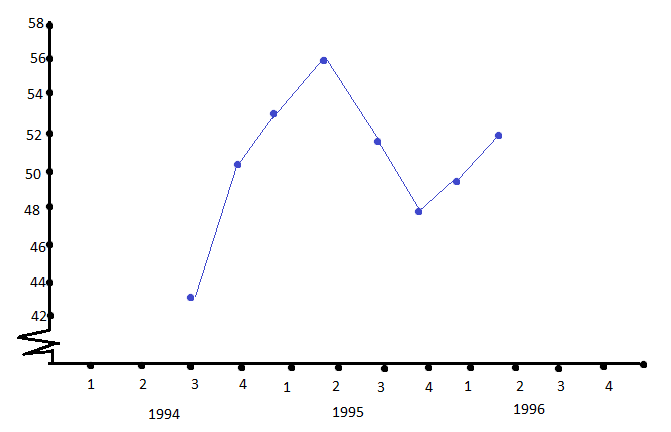# ISC Class 11 Maths Specimen Question Paper 2018 With Answers - Free Sample Paper PDF Download

ISC Class 11 Maths 2018 Specimen Question Paper with Solutions are available at BYJU’S, that can be accessed anytime by downloading a pdf. These solutions of ISC Maths Class 11 specimen papers are given by the expert faculty, hence they are accurate and efficient. All the important methods of solving maths problems are covered here for each of these questions.

Class 11 Students can download and practise board papers of ISC Class 11 Maths as a part of their examination preparation. This will help them in facing a variety of problems and methods of solving them so that they can improve their analytical skills to reach the right approach.

## ISC Class 11 Maths Specimen Question Paper 2018 with Solutions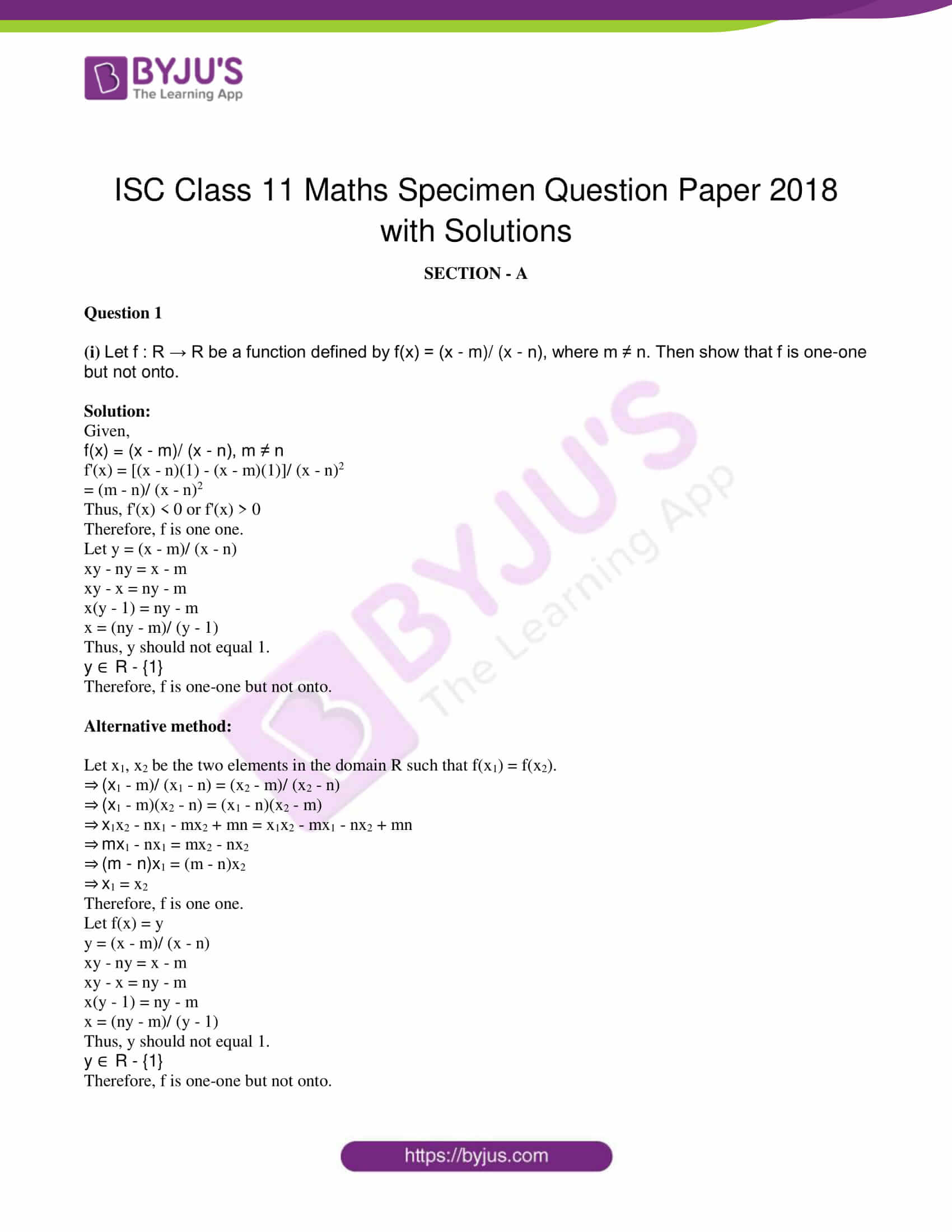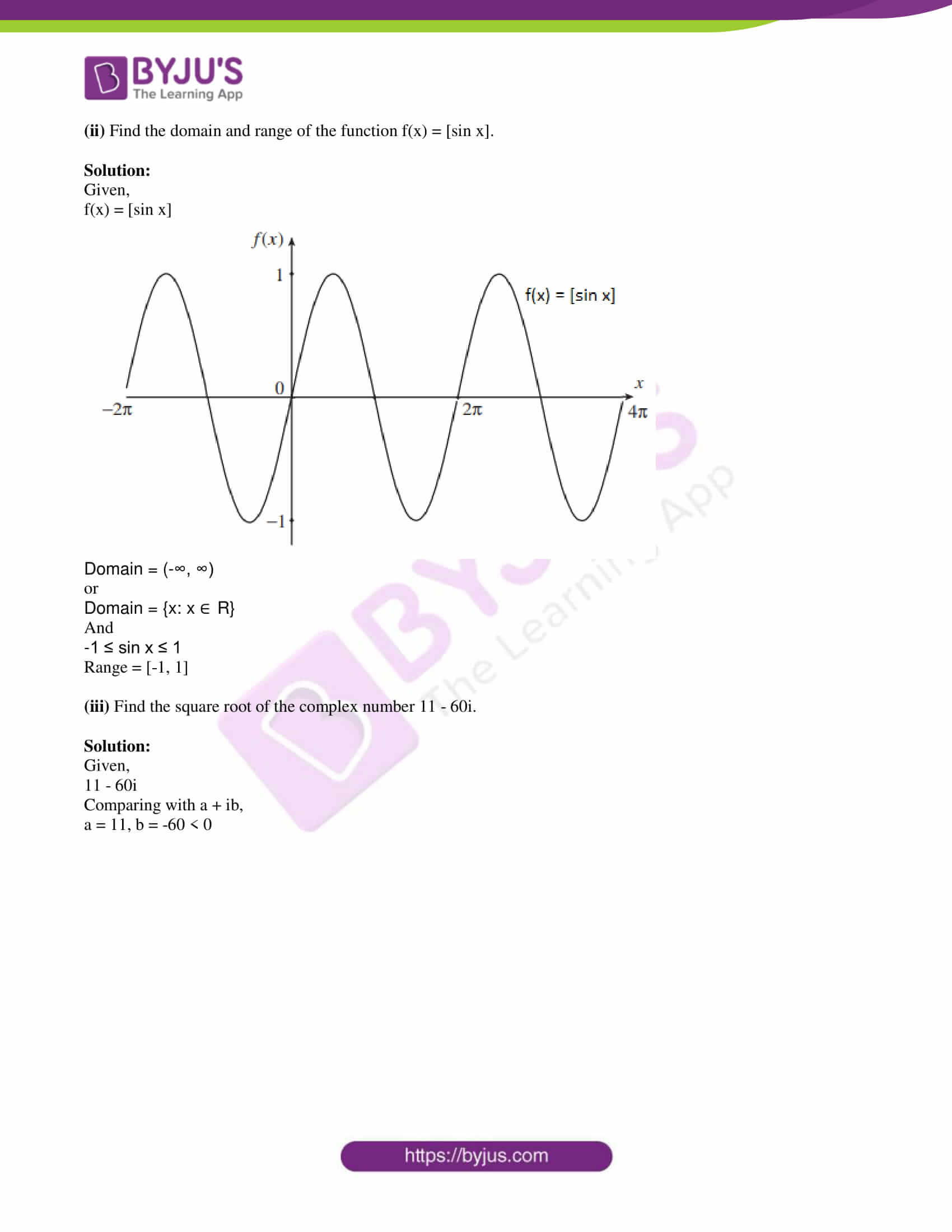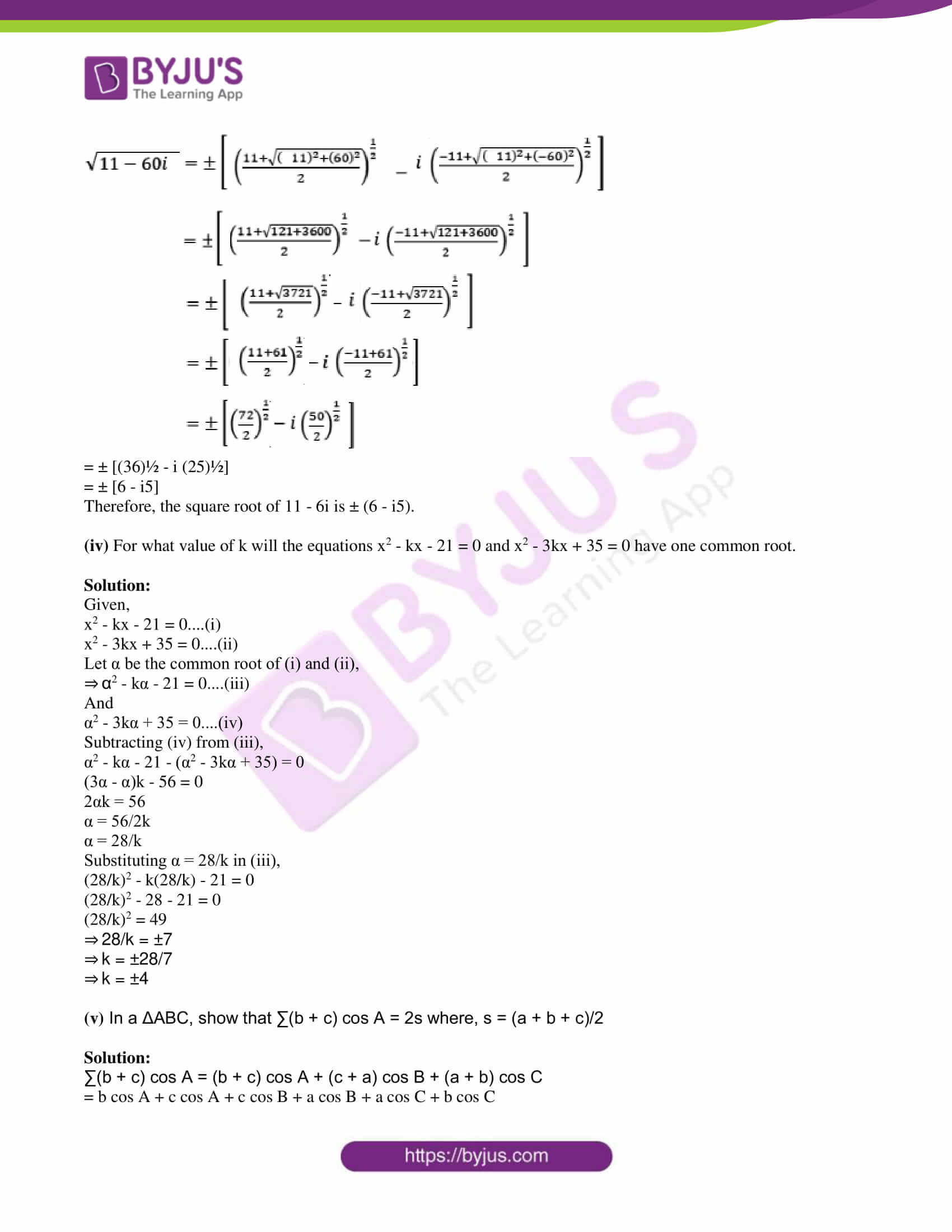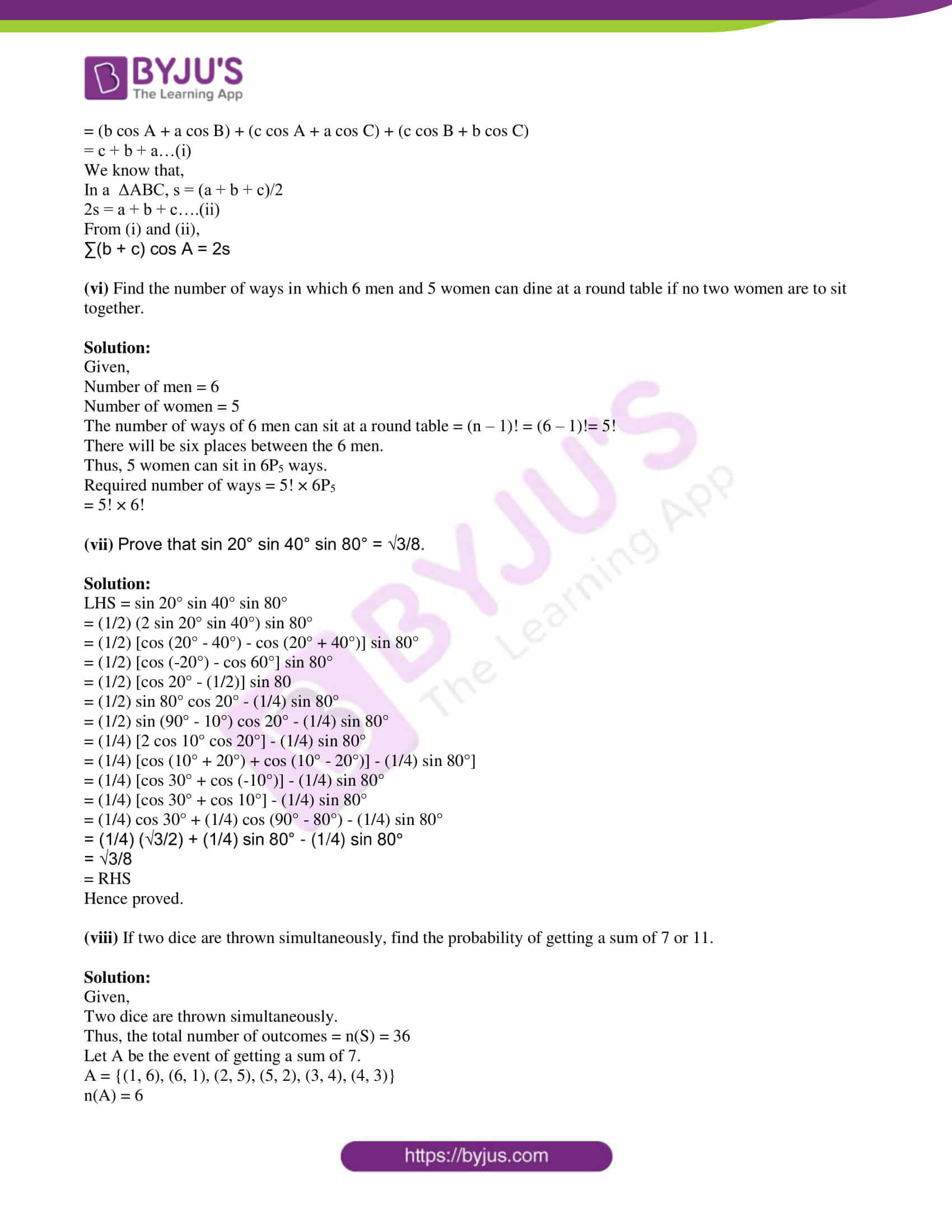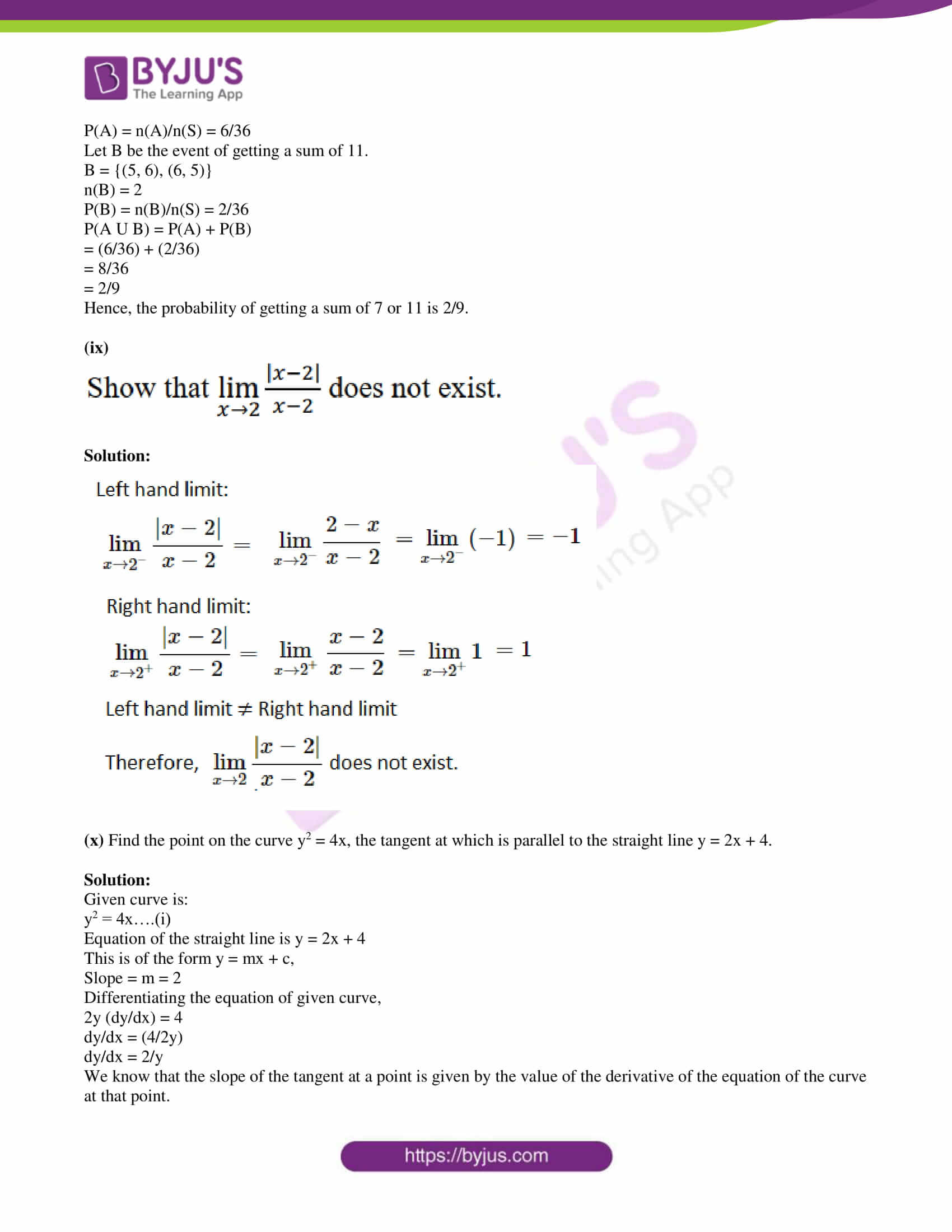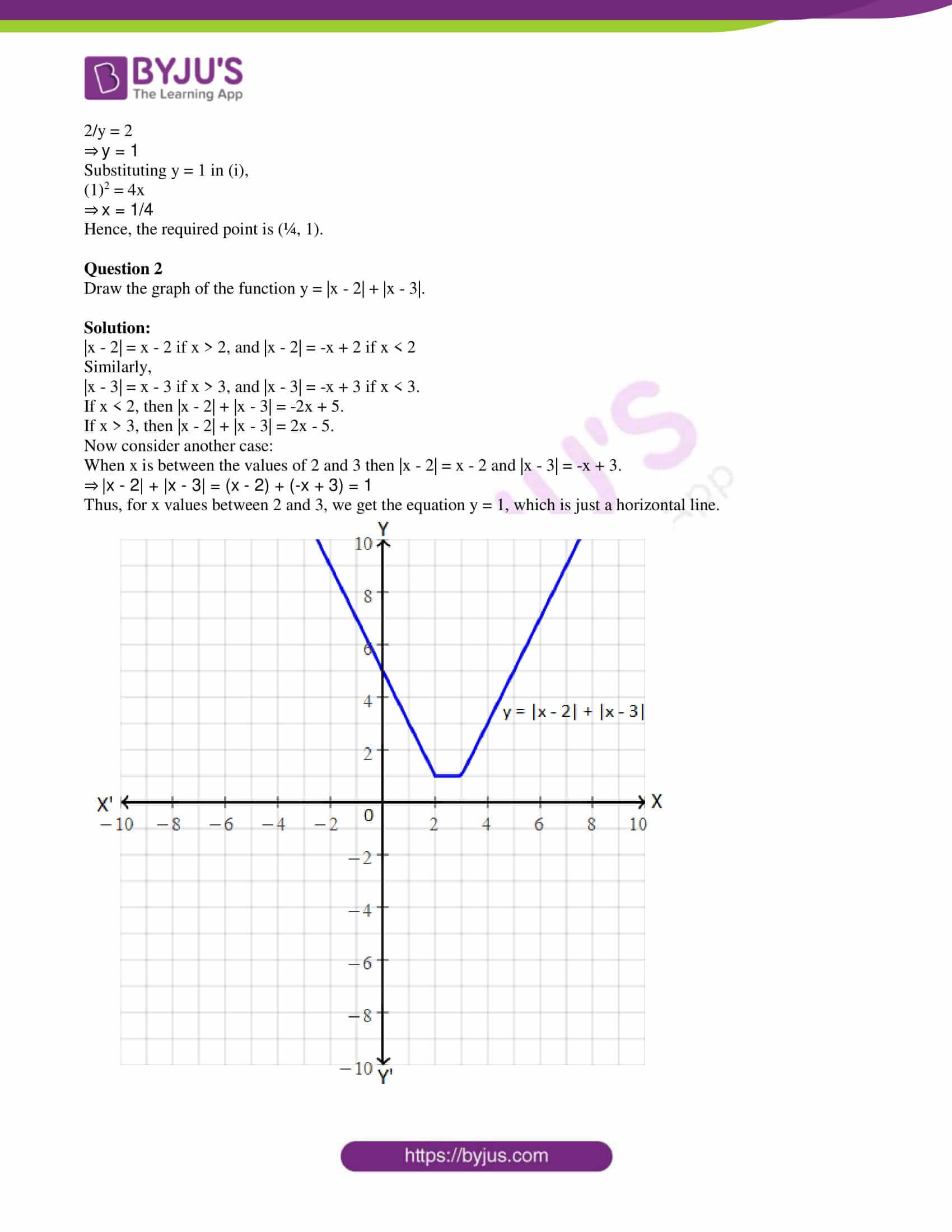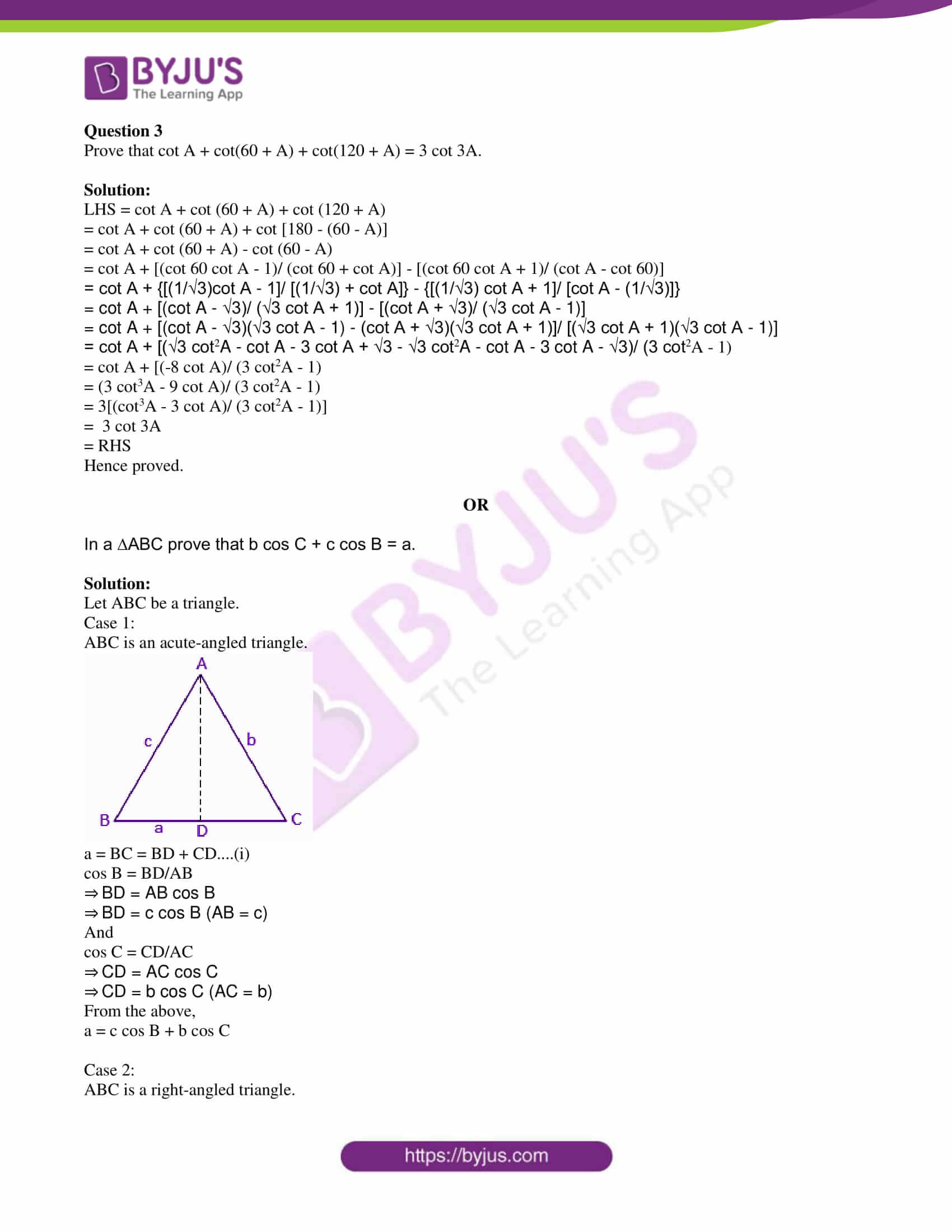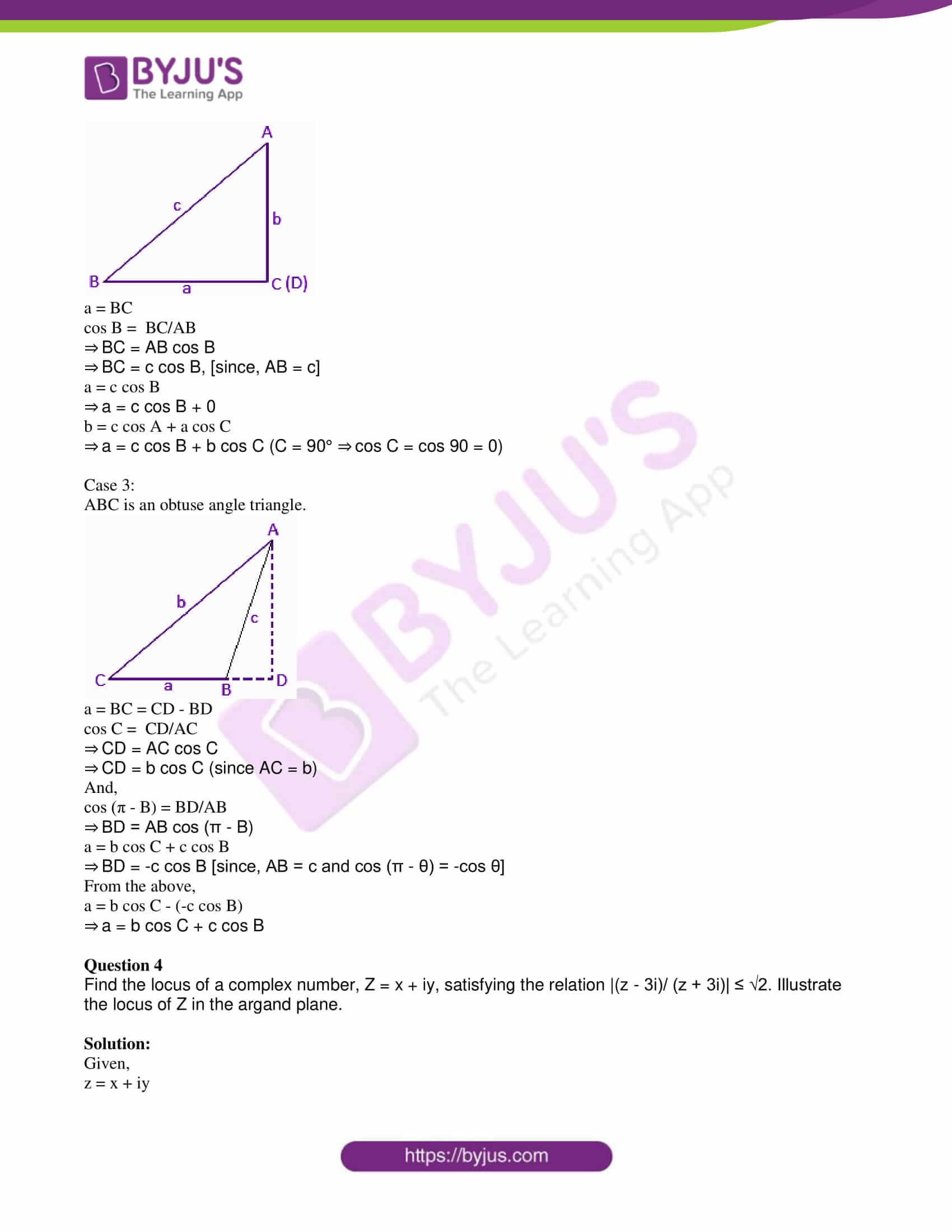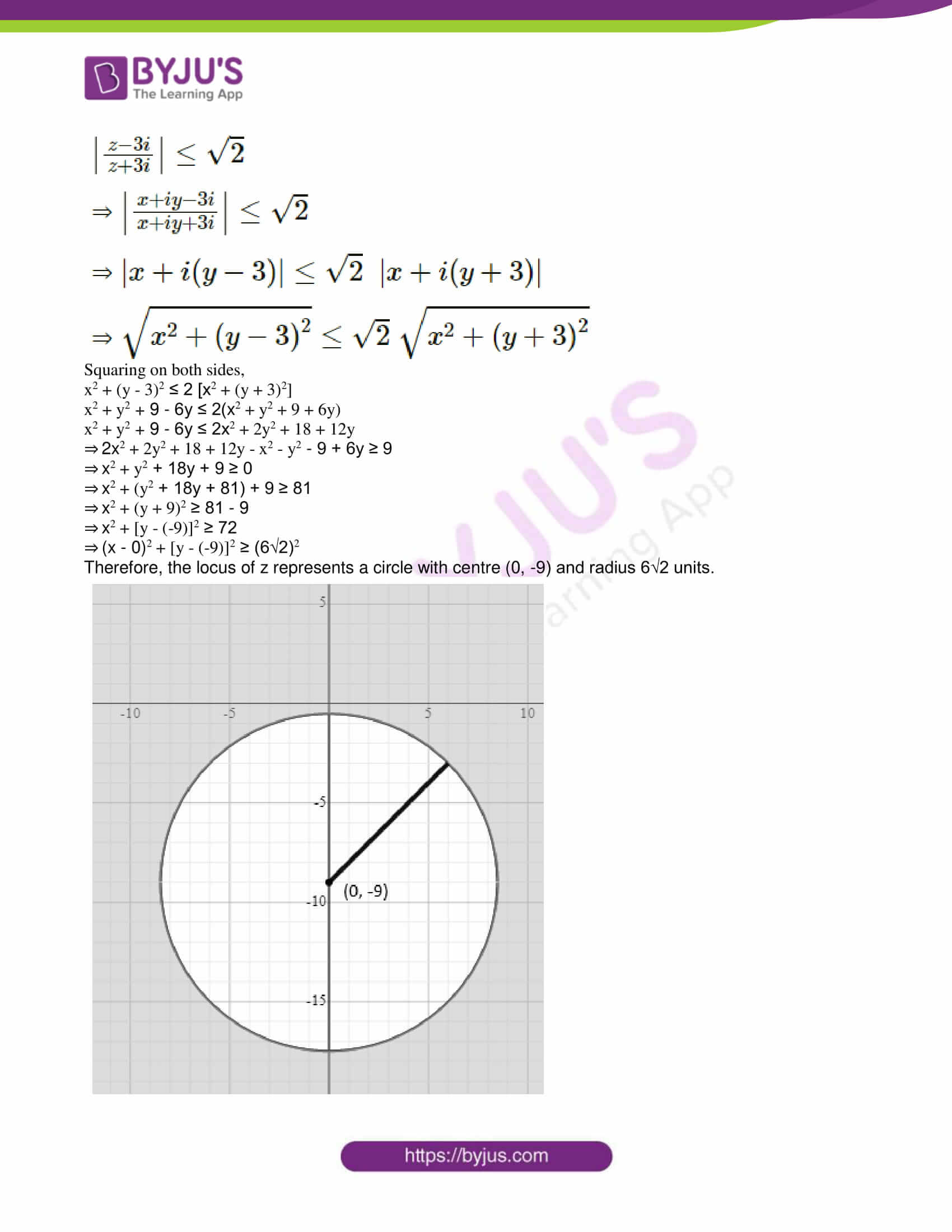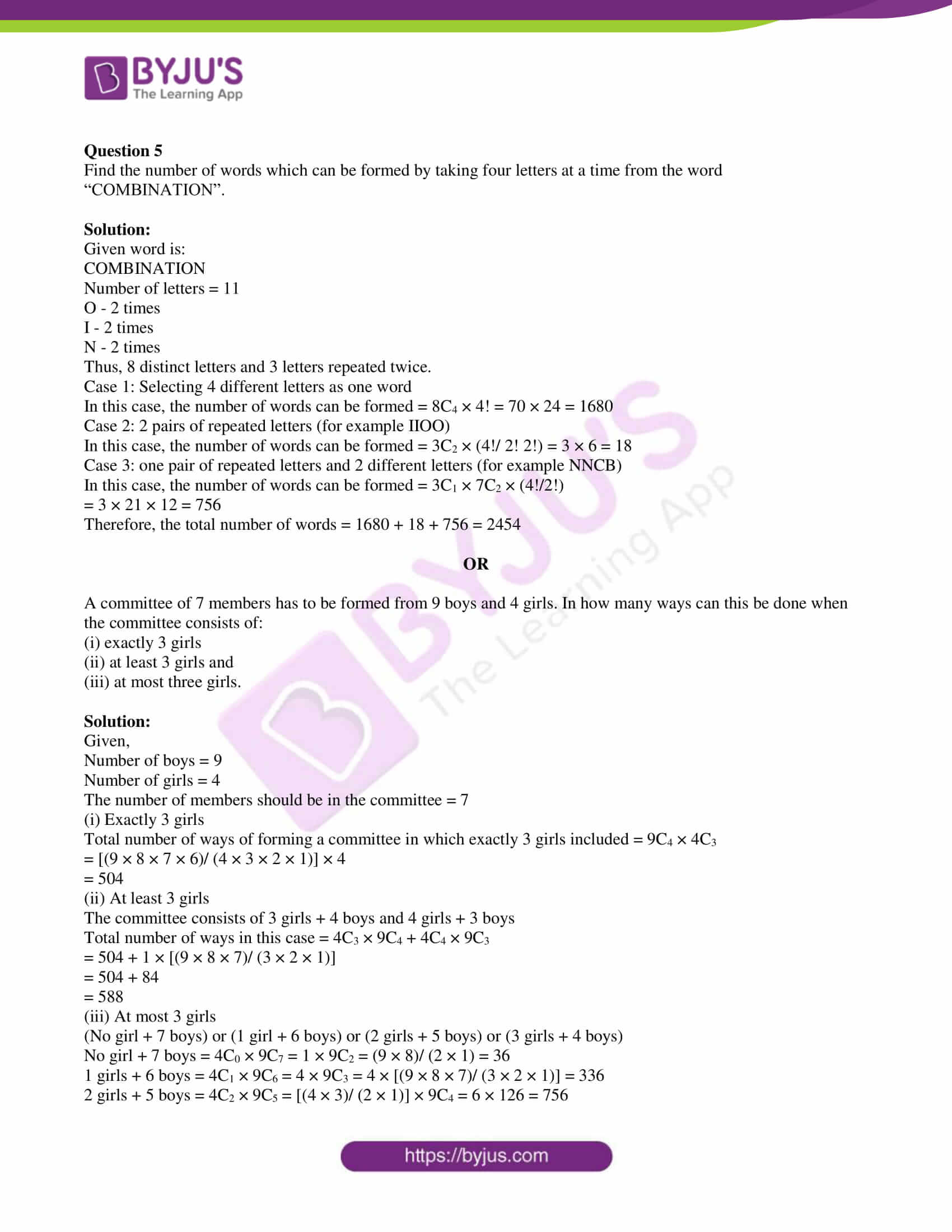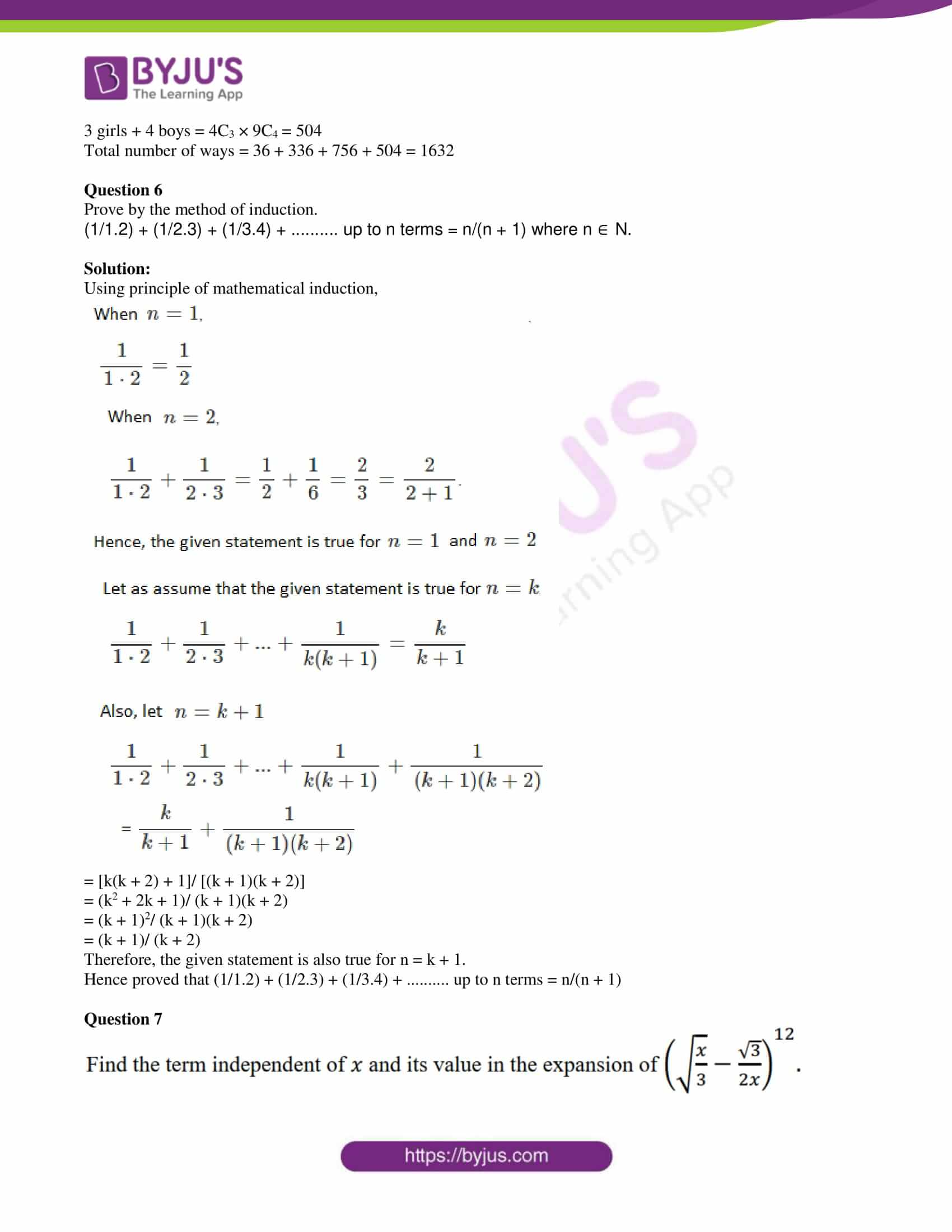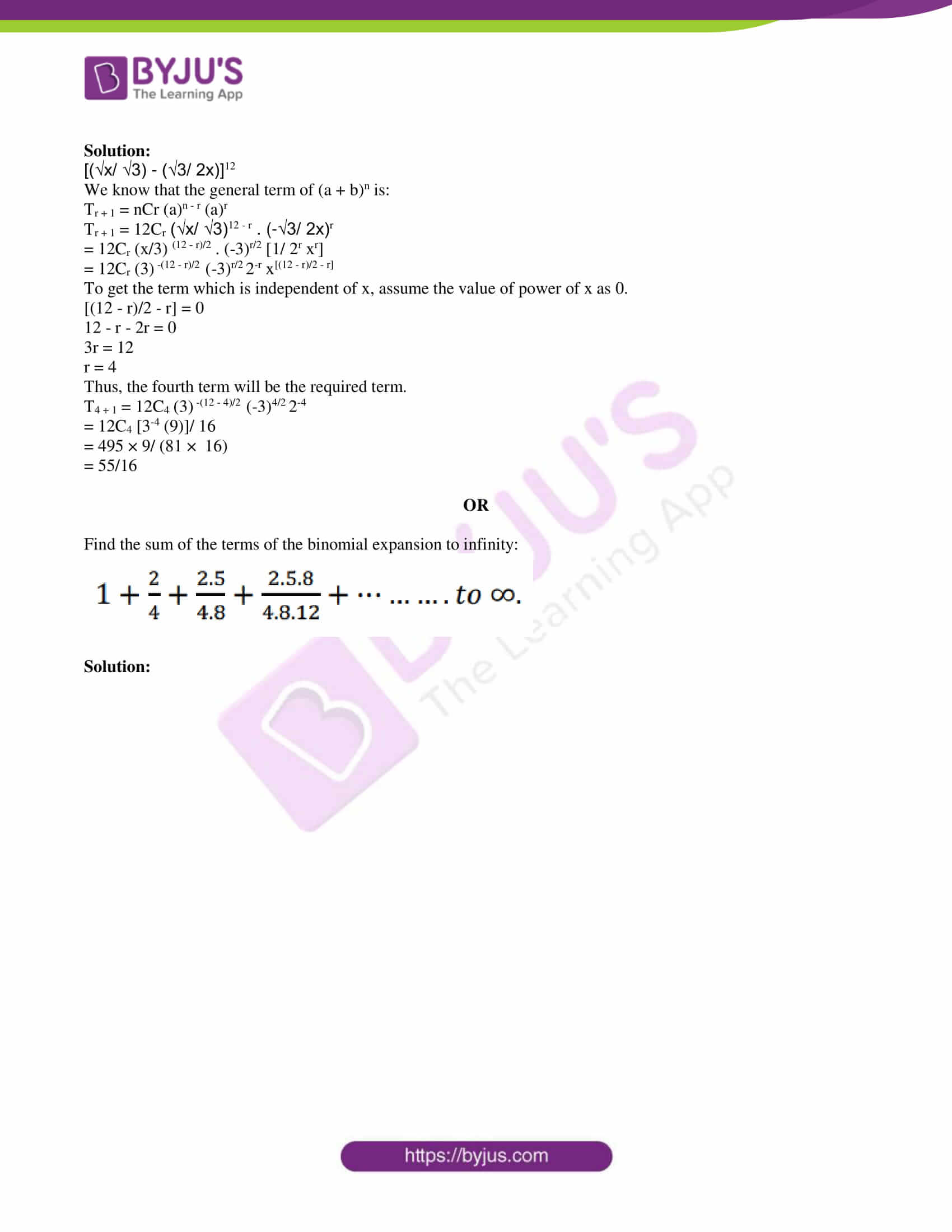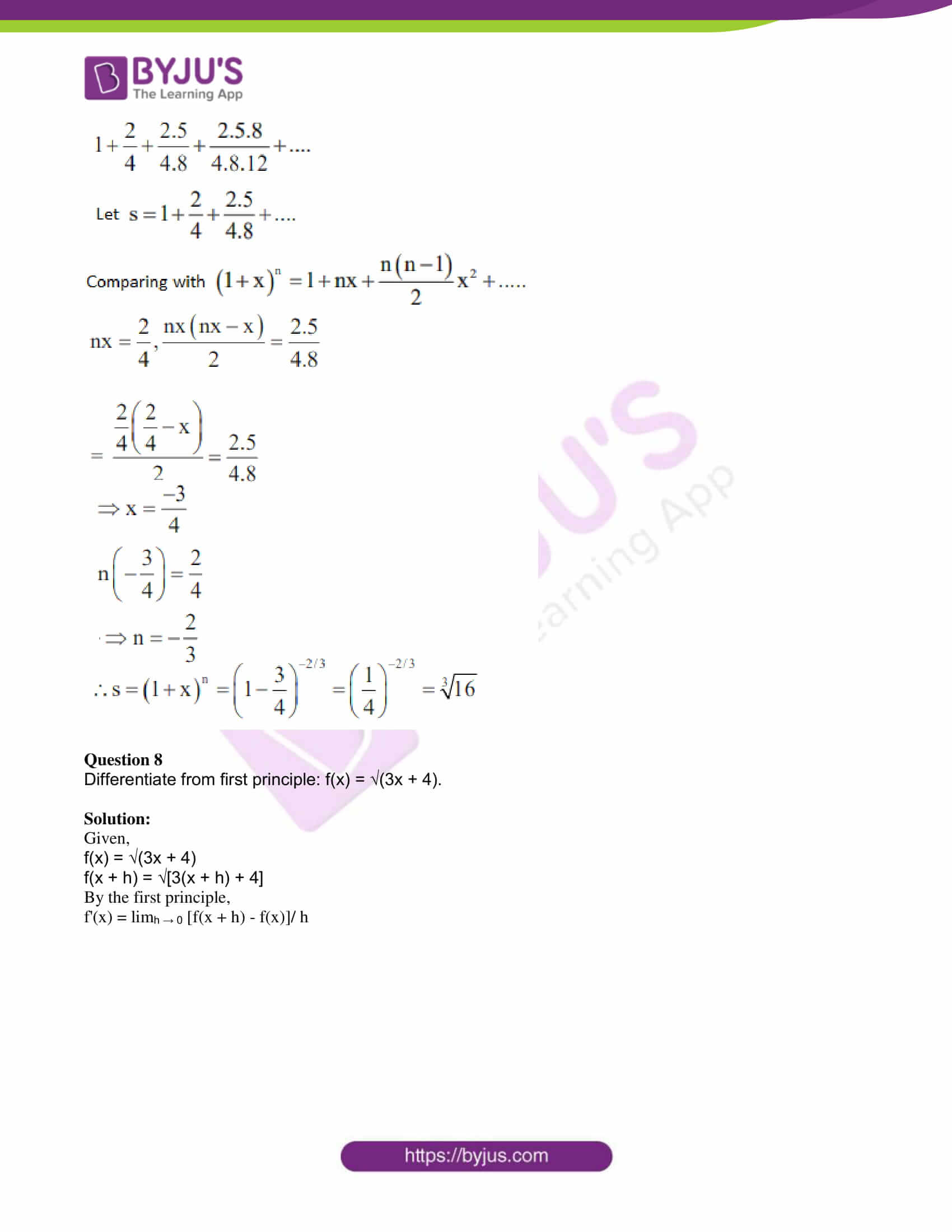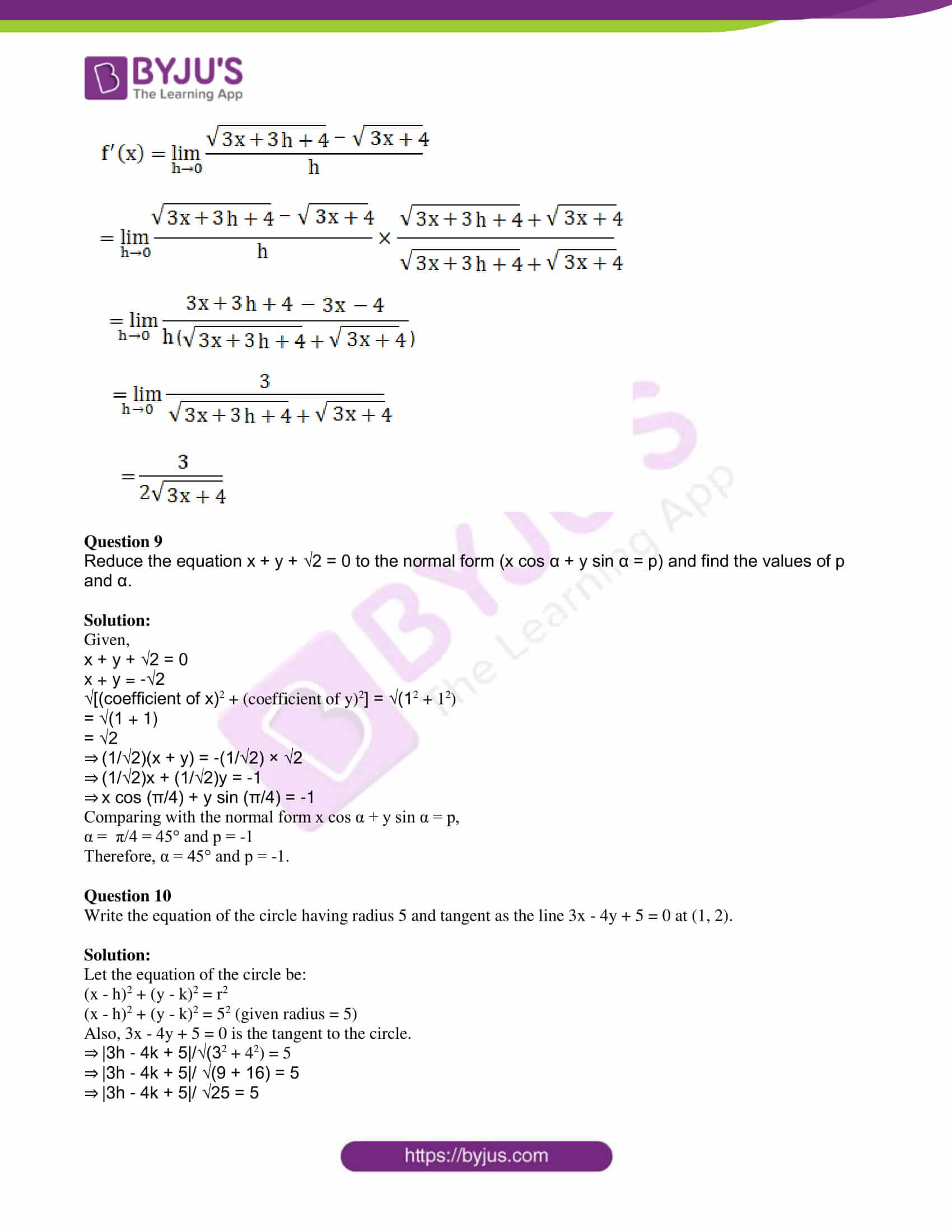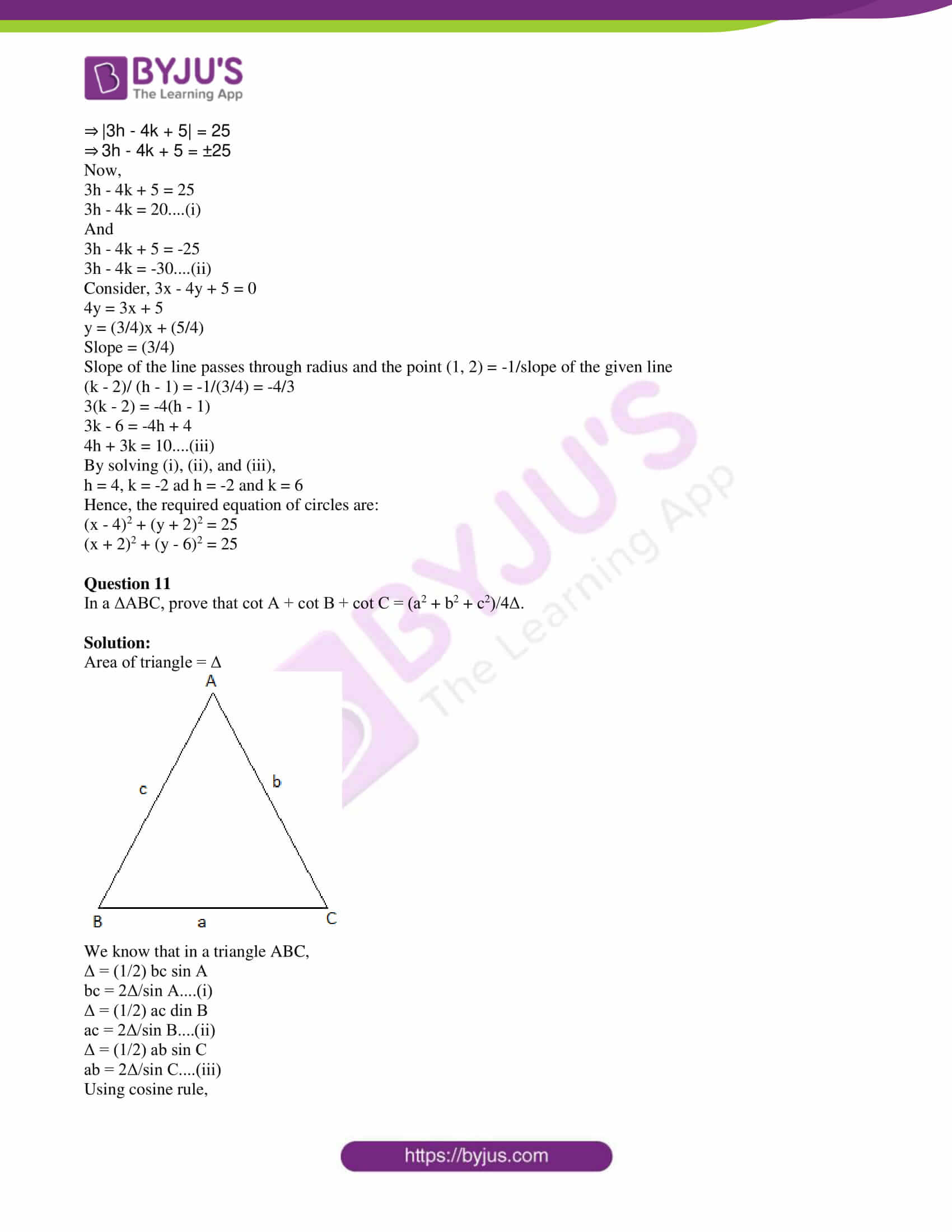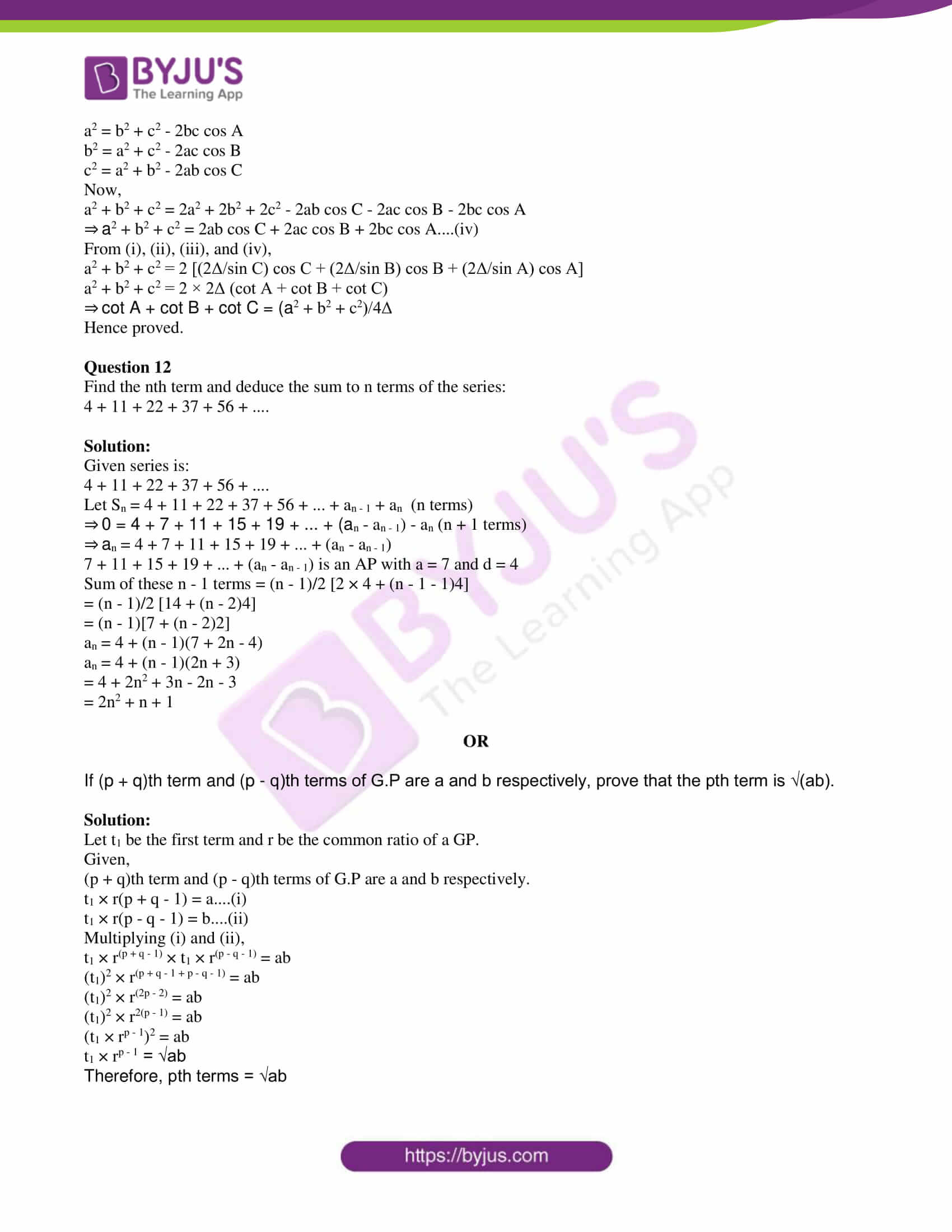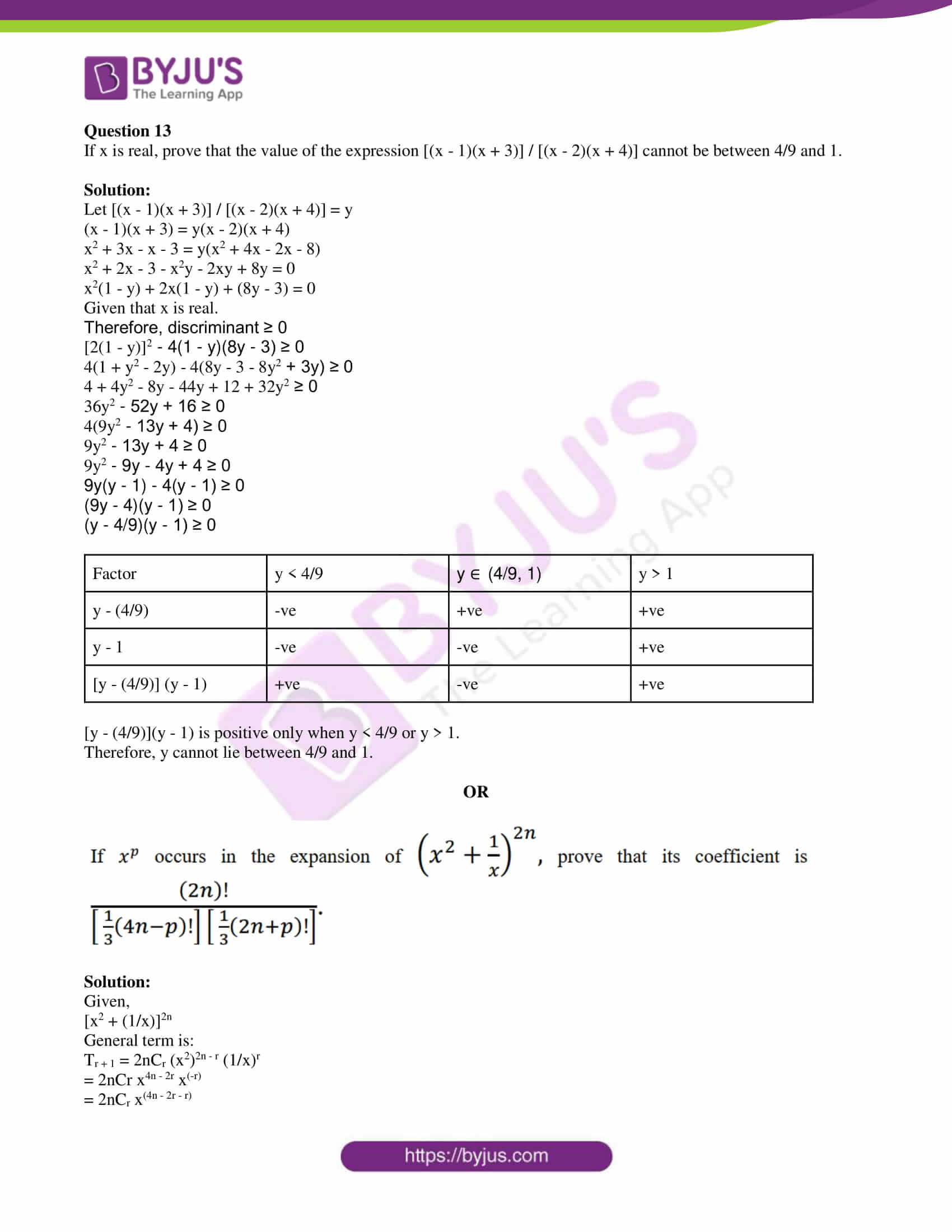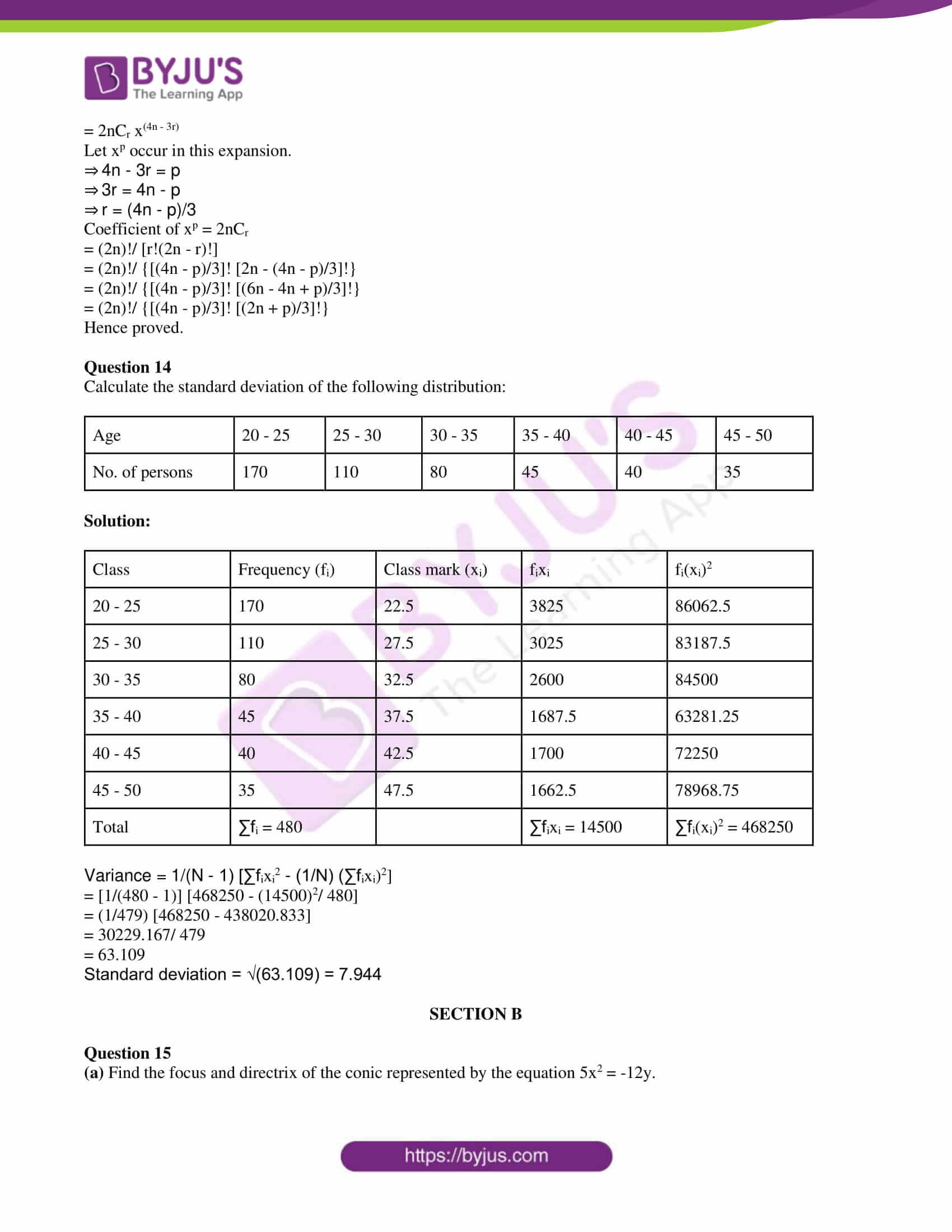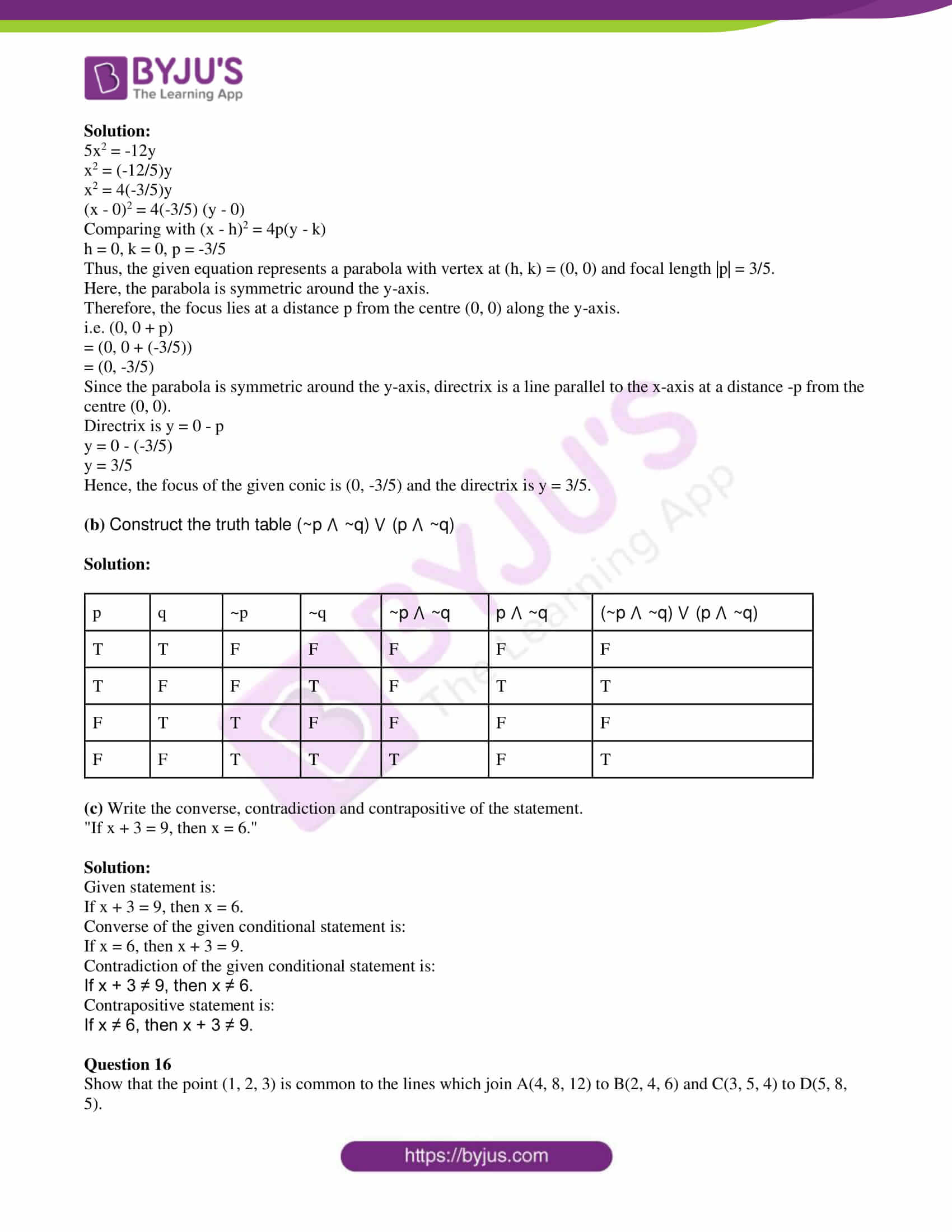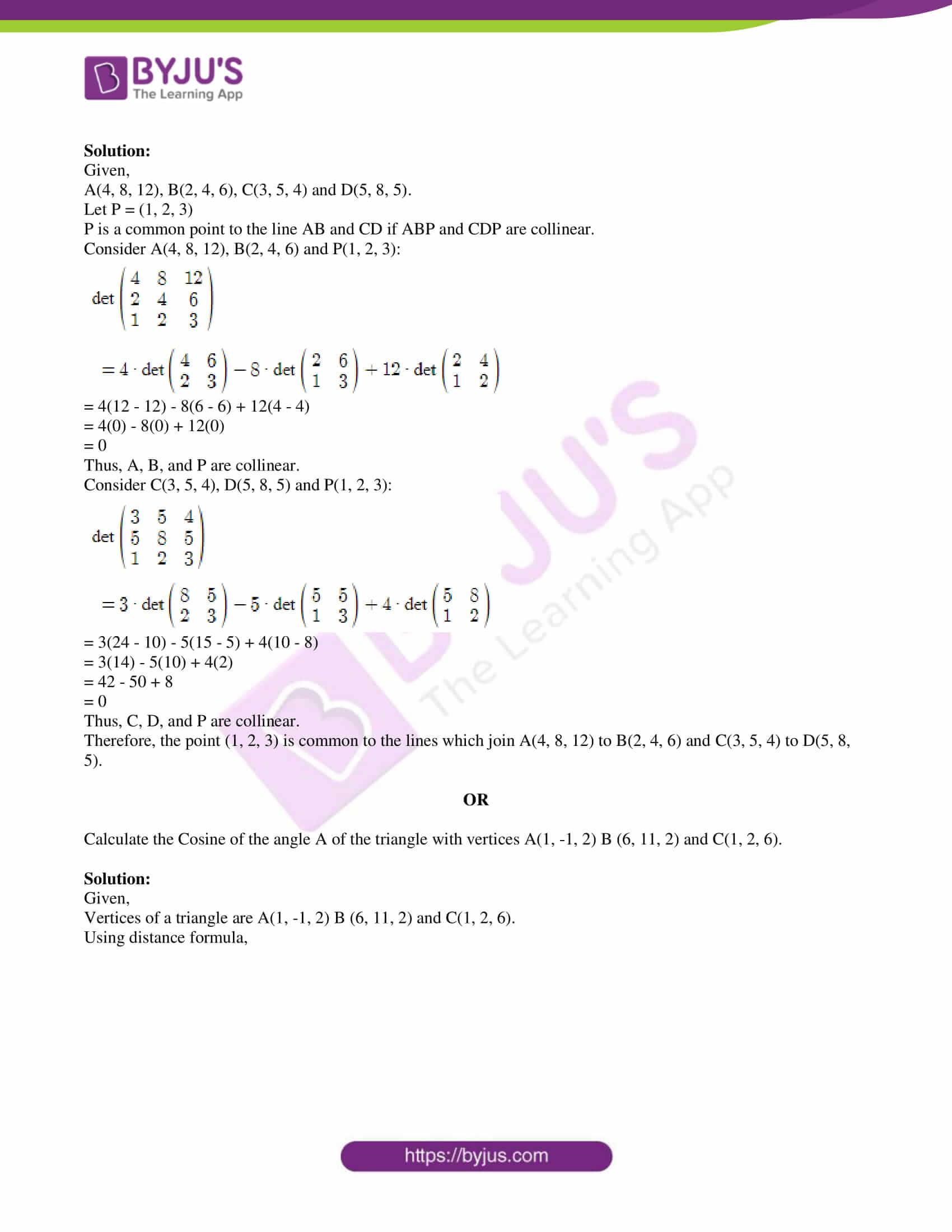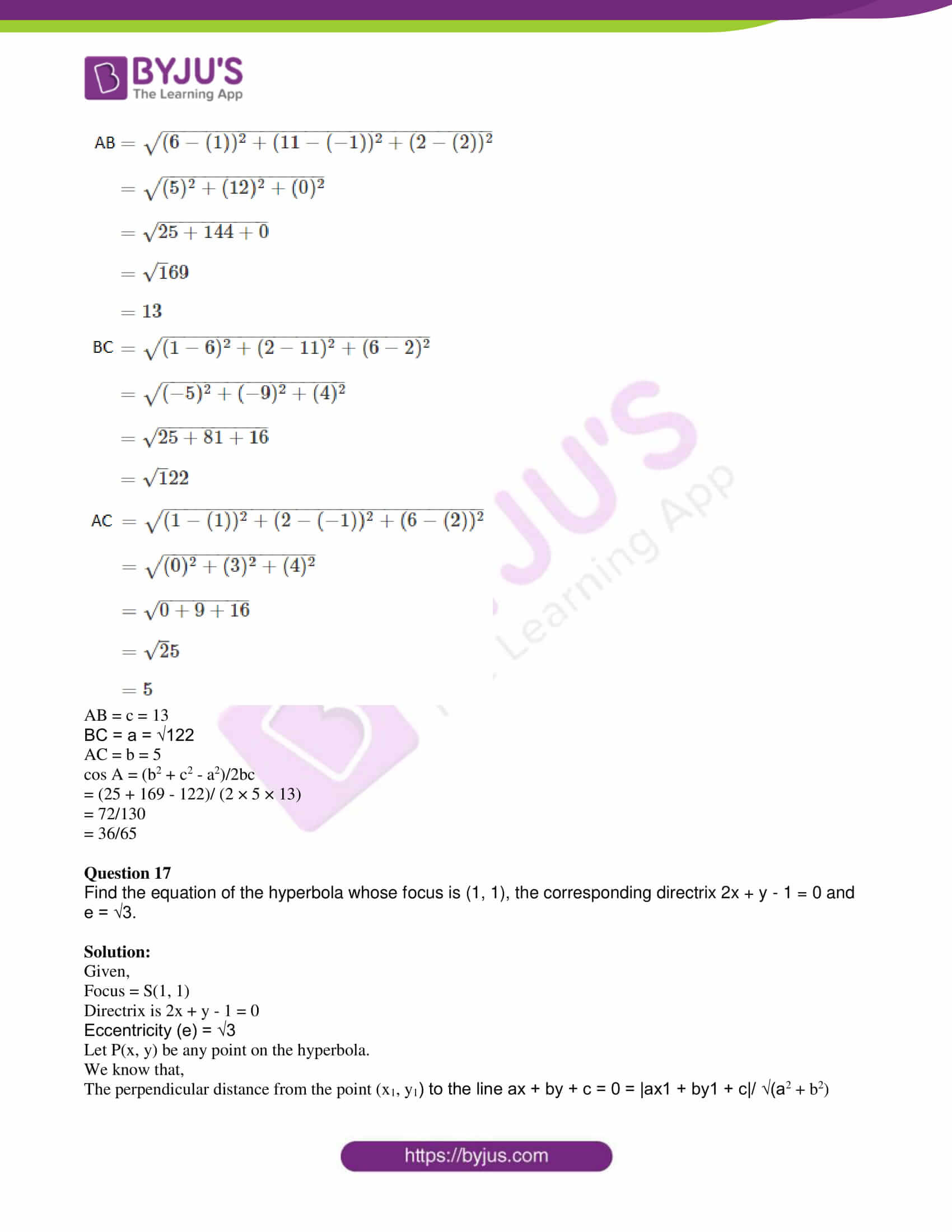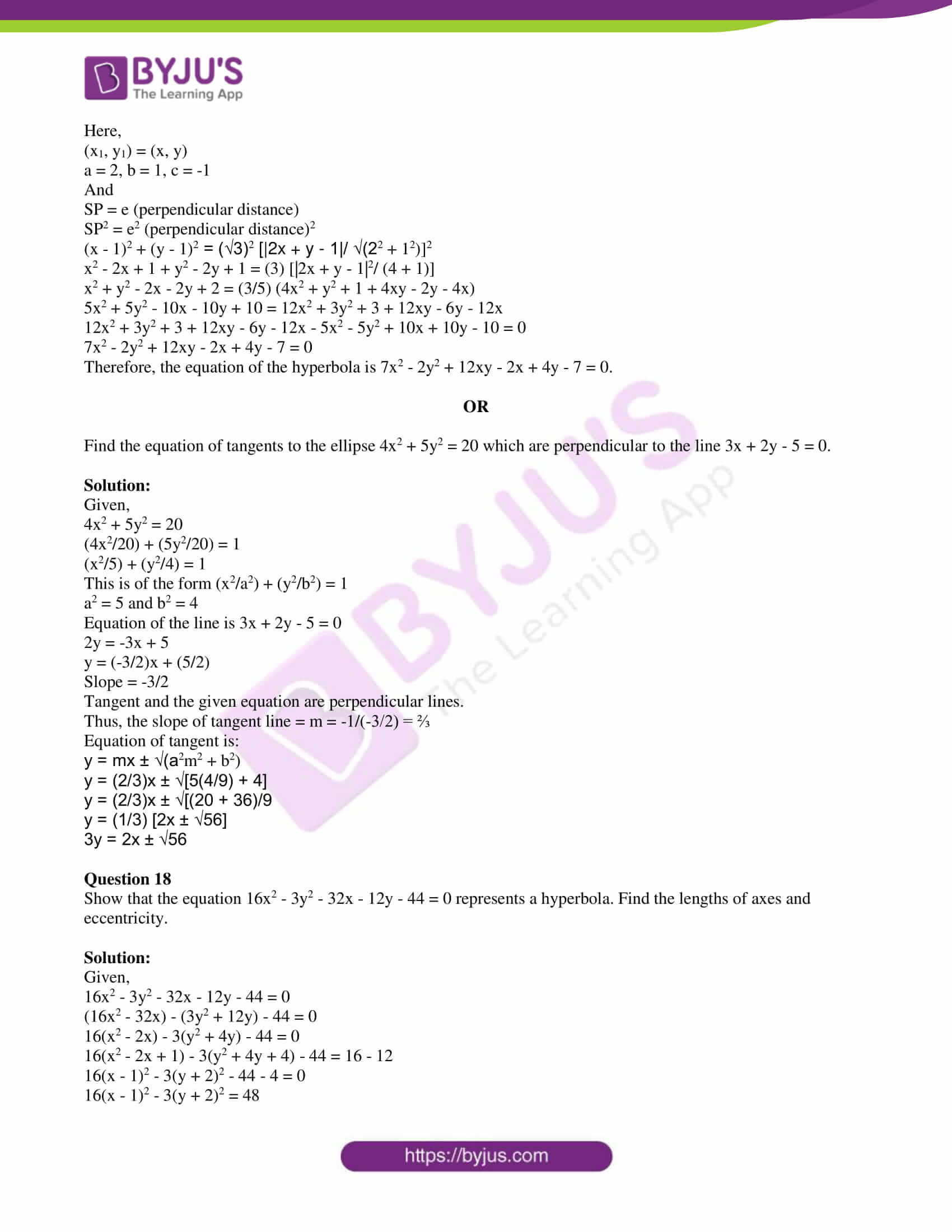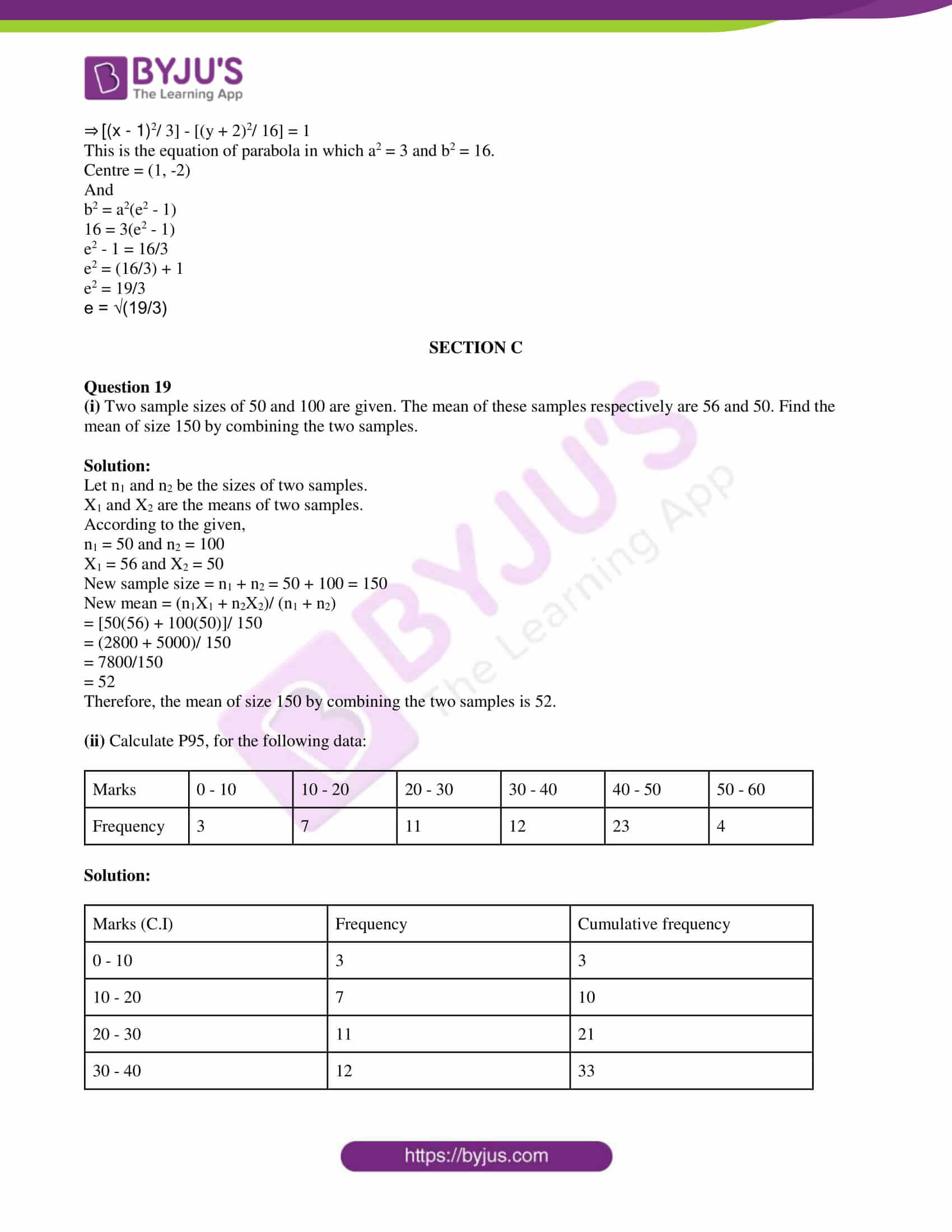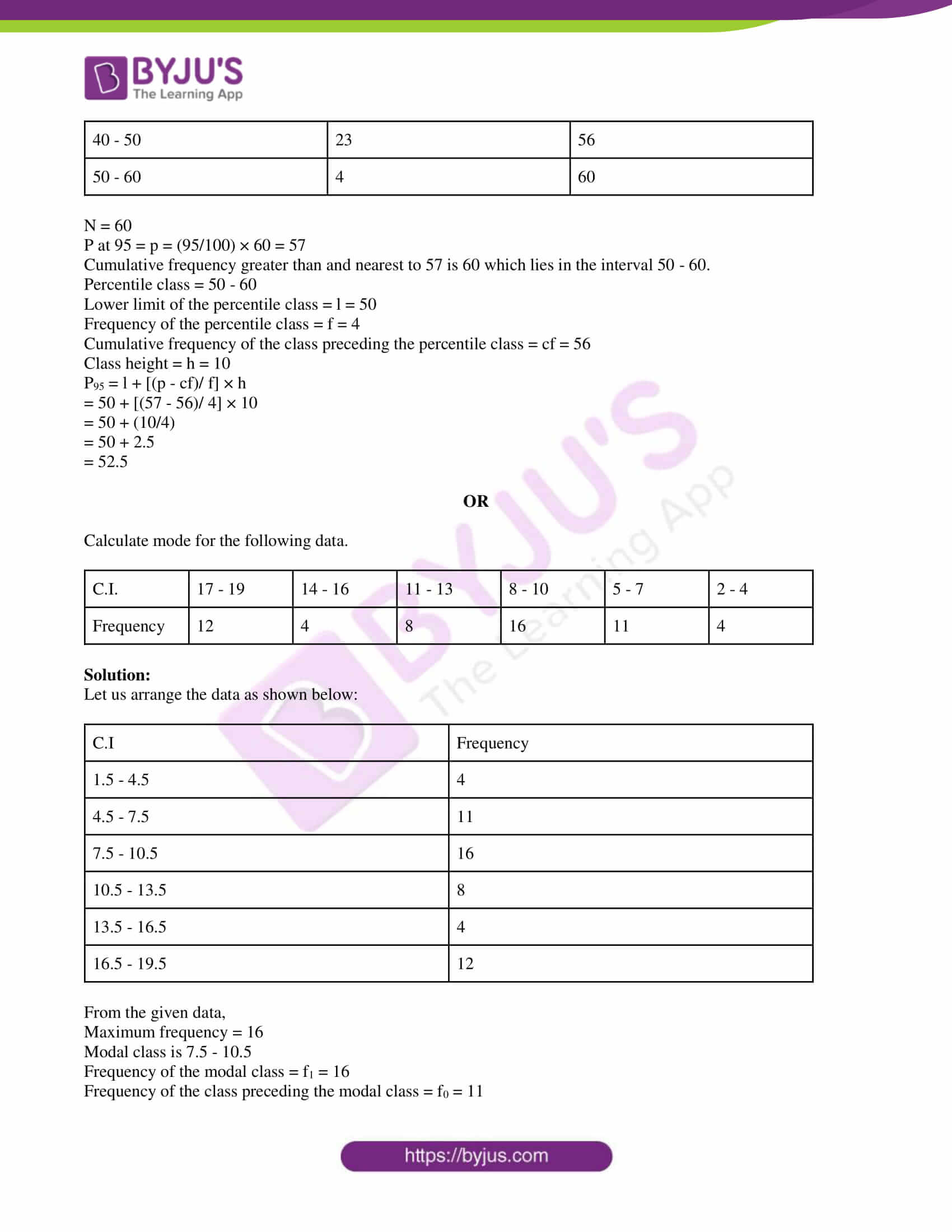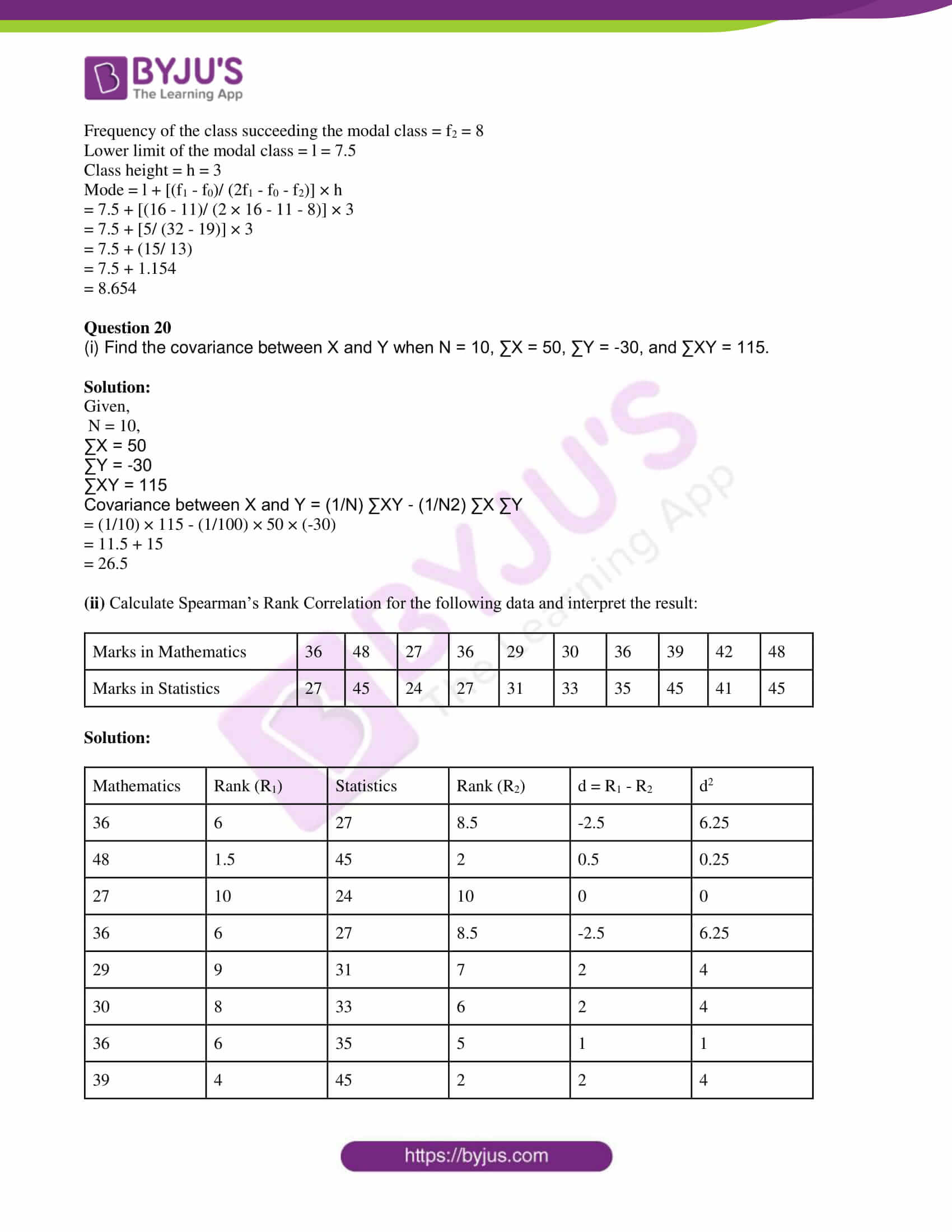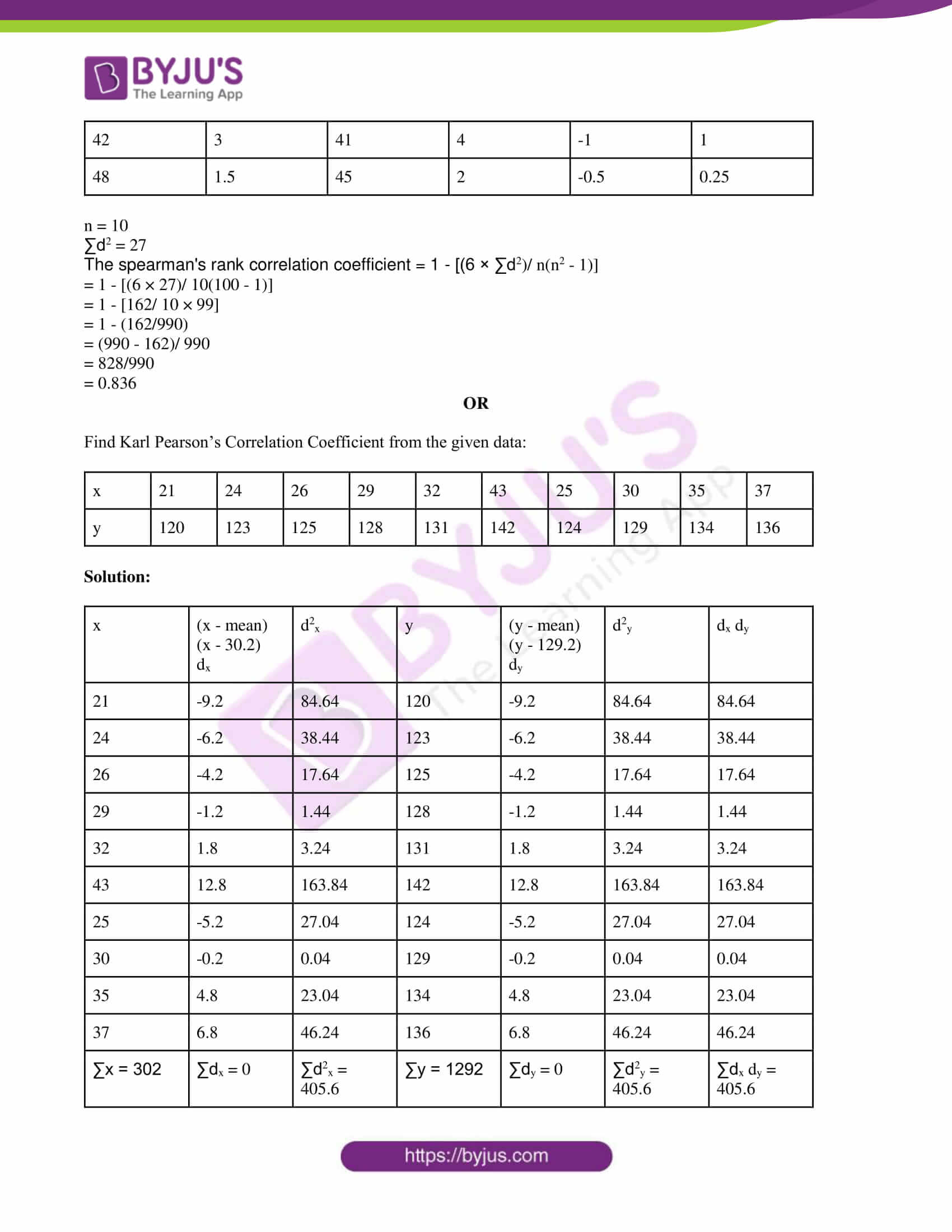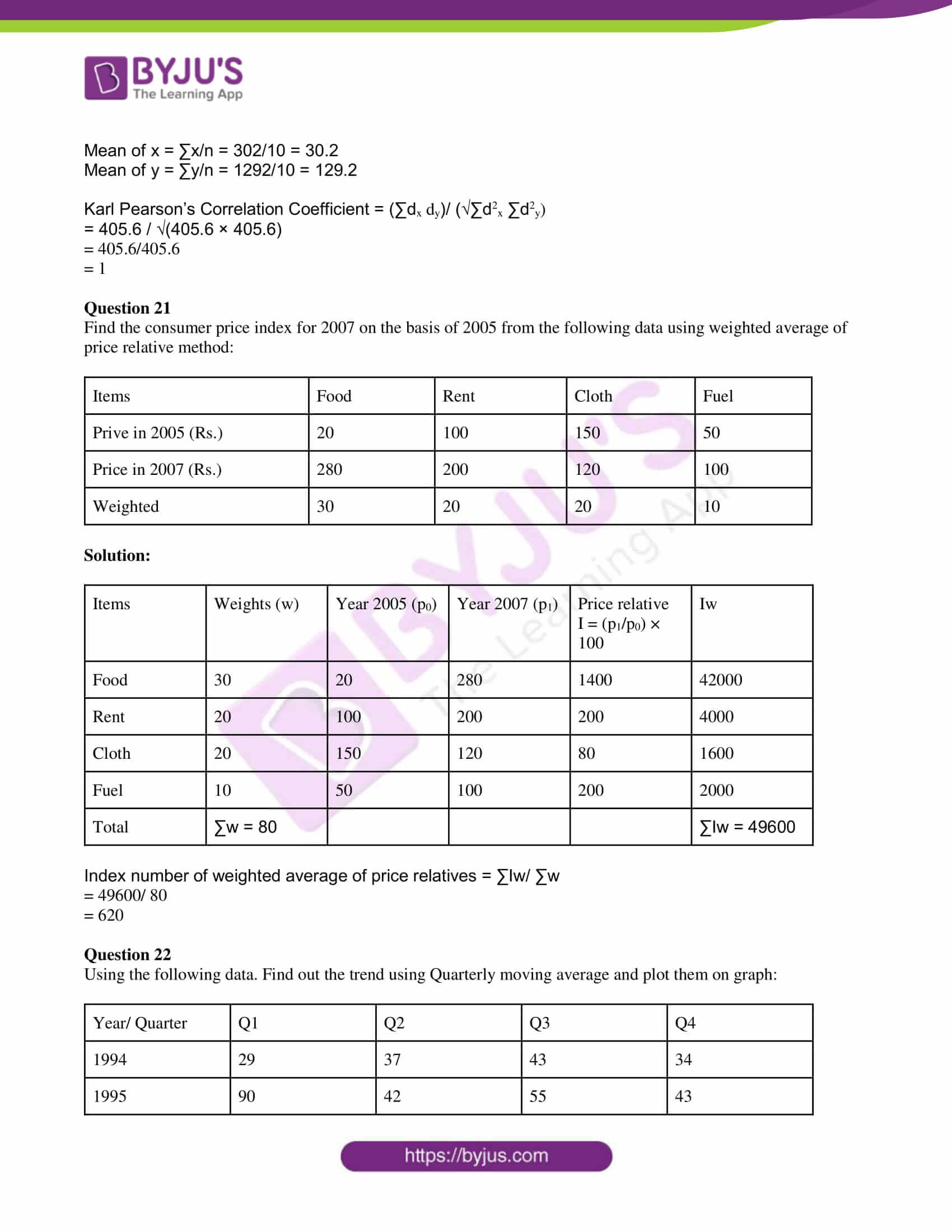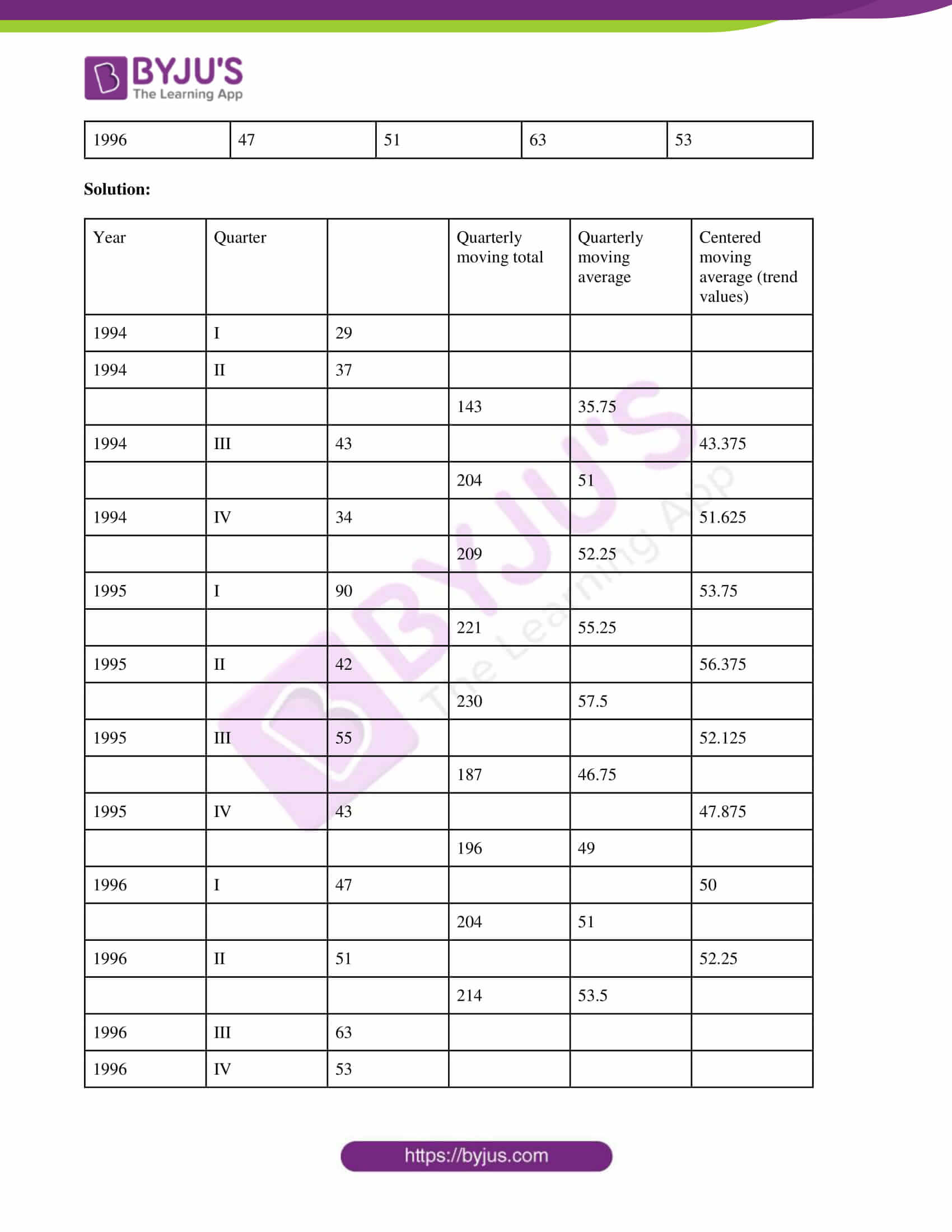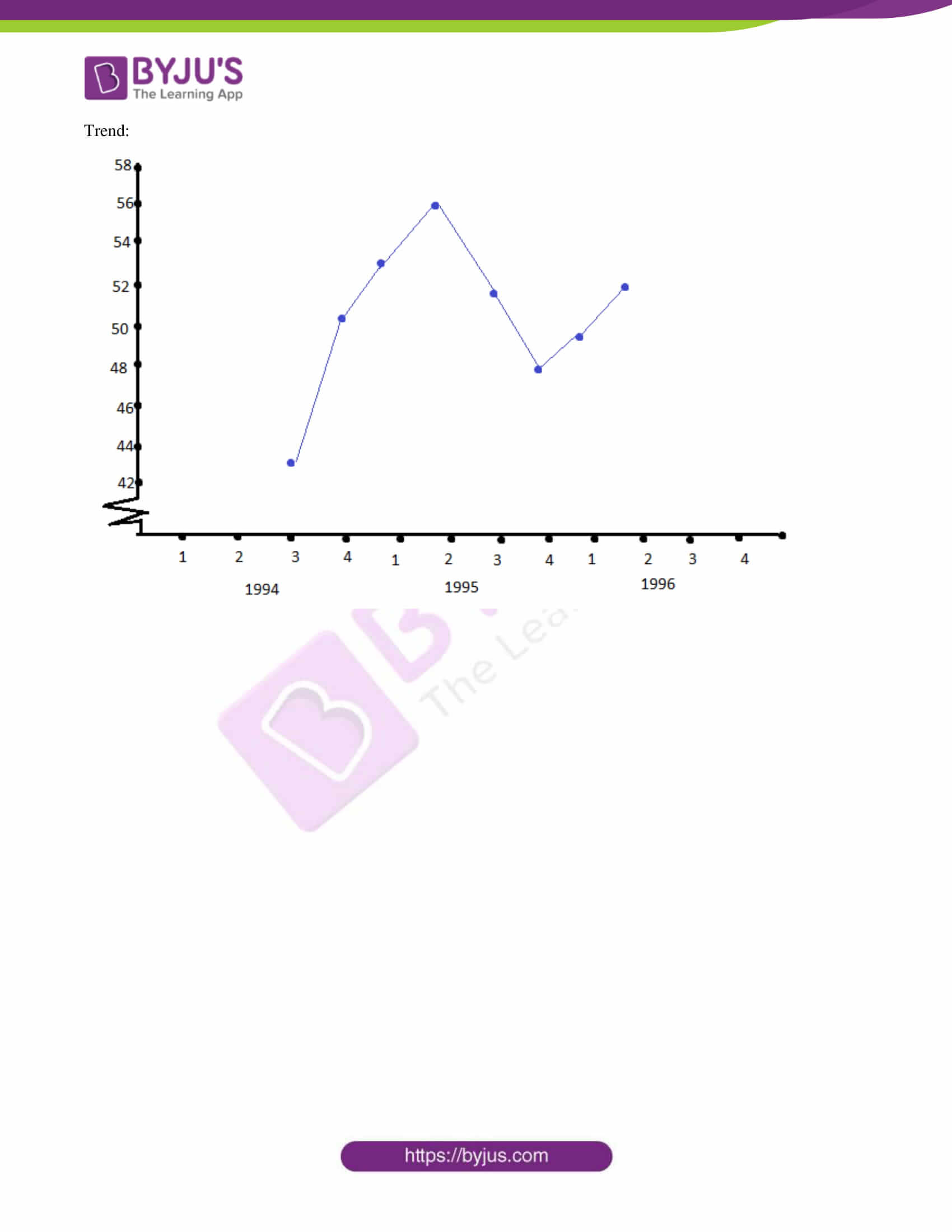SECTION – A

Question 1

(i) Let f : R → R be a function defined by f(x) = (x – m)/ (x – n), where m ≠ n. Then show that f is one-one but not onto.

Solution:

Given,

f(x) = (x – m)/ (x – n), m ≠ n

f'(x) = [(x – n)(1) – (x – m)(1)]/ (x – n)2

= (m – n)/ (x – n)2

Thus, f'(x) < 0 or f'(x) > 0

Therefore, f is one one.

Let y = (x – m)/ (x – n)

xy – ny = x – m

xy – x = ny – m

x(y – 1) = ny – m

x = (ny – m)/ (y – 1)

Thus, y should not equal 1.

y ∈ R – {1}

Therefore, f is one-one but not onto.

Alternative method:

Let x1, x2 be the two elements in the domain R such that f(x1) = f(x2).

⇒ (x1 – m)/ (x1 – n) = (x2 – m)/ (x2 – n)

⇒ (x1 – m)(x2 – n) = (x1 – n)(x2 – m)

⇒ x1x2 – nx1 – mx2 + mn = x1x2 – mx1 – nx2 + mn

⇒ mx1 – nx1 = mx2 – nx2

⇒ (m – n)x1 = (m – n)x2

⇒ x1 = x2

Therefore, f is one one.

Let f(x) = y

y = (x – m)/ (x – n)

xy – ny = x – m

xy – x = ny – m

x(y – 1) = ny – m

x = (ny – m)/ (y – 1)

Thus, y should not equal 1.

y ∈ R – {1}

Therefore, f is one-one but not onto.

(ii) Find the domain and range of the function f(x) = [sin x].

Solution:

Given,

f(x) = [sin x]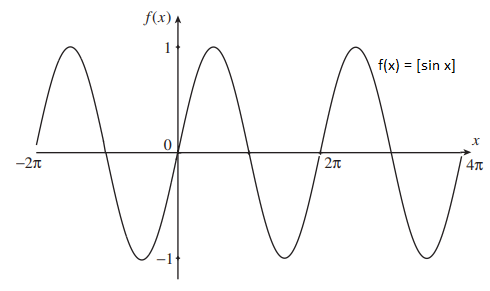Domain = (-∞, ∞)

or

Domain = {x: x ∈ R}

And

-1 ≤ sin x ≤ 1

Range = [-1, 1]

(iii) Find the square root of the complex number 11 – 60i.

Solution:

Given,

11 – 60i

Comparing with a + ib,

a = 11, b = -60 < 0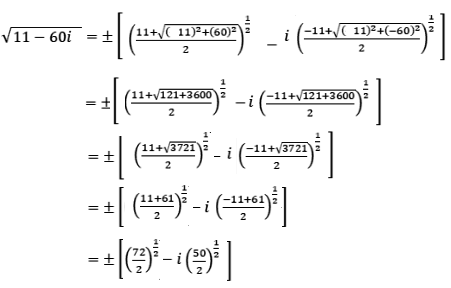= ± [(36)½ – i (25)½]

= ± [6 – i5]

Therefore, the square root of 11 – 6i is ± (6 – i5).

(iv) For what value of k will the equations x2 – kx – 21 = 0 and x2 – 3kx + 35 = 0 have one common root.

Solution:

Given,

x2 – kx – 21 = 0….(i)

x2 – 3kx + 35 = 0….(ii)

Let α be the common root of (i) and (ii),

⇒ α2 – kα – 21 = 0….(iii)

And

α2 – 3kα + 35 = 0….(iv)

Subtracting (iv) from (iii),

α2 – kα – 21 – (α2 – 3kα + 35) = 0

(3α – α)k – 56 = 0

2αk = 56

α = 56/2k

α = 28/k

Substituting α = 28/k in (iii),

(28/k)2 – k(28/k) – 21 = 0

(28/k)2 – 28 – 21 = 0

(28/k)2 = 49

⇒ 28/k = ±7

⇒ k = ±28/7

⇒ k = ±4

(v) In a ΔABC, show that ∑(b + c) cos A = 2s where, s = (a + b + c)/2

Solution:

∑(b + c) cos A = (b + c) cos A + (c + a) cos B + (a + b) cos C

= b cos A + c cos A + c cos B + a cos B + a cos C + b cos C

= (b cos A + a cos B) + (c cos A + a cos C) + (c cos B + b cos C)

= c + b + a…(i)

We know that,

In a ΔABC, s = (a + b + c)/2

2s = a + b + c….(ii)

From (i) and (ii),

∑(b + c) cos A = 2s

(vi) Find the number of ways in which 6 men and 5 women can dine at a round table if no two women are to sit together.

Solution:

Given,

Number of men = 6

Number of women = 5

The number of ways of 6 men can sit at a round table = (n – 1)! = (6 – 1)!= 5!

There will be six places between the 6 men.

Thus, 5 women can sit in 6P5 ways.

Required number of ways = 5! × 6P5

= 5! × 6!

(vii) Prove that sin 20° sin 40° sin 80° = √3/8.

Solution:

LHS = sin 20° sin 40° sin 80°

= (1/2) (2 sin 20° sin 40°) sin 80°

= (1/2) [cos (20° – 40°) – cos (20° + 40°)] sin 80°

= (1/2) [cos (-20°) – cos 60°] sin 80°

= (1/2) [cos 20° – (1/2)] sin 80

= (1/2) sin 80° cos 20° – (1/4) sin 80°

= (1/2) sin (90° – 10°) cos 20° – (1/4) sin 80°

= (1/4) [2 cos 10° cos 20°] – (1/4) sin 80°

= (1/4) [cos (10° + 20°) + cos (10° – 20°)] – (1/4) sin 80°]

= (1/4) [cos 30° + cos (-10°)] – (1/4) sin 80°

= (1/4) [cos 30° + cos 10°] – (1/4) sin 80°

= (1/4) cos 30° + (1/4) cos (90° – 80°) – (1/4) sin 80°

= (1/4) (√3/2) + (1/4) sin 80° – (1/4) sin 80°

= √3/8

= RHS

Hence proved.

(viii) If two dice are thrown simultaneously, find the probability of getting a sum of 7 or 11.

Solution:

Given,

Two dice are thrown simultaneously.

Thus, the total number of outcomes = n(S) = 36

Let A be the event of getting a sum of 7.

A = {(1, 6), (6, 1), (2, 5), (5, 2), (3, 4), (4, 3)}

n(A) = 6

P(A) = n(A)/n(S) = 6/36

Let B be the event of getting a sum of 11.

B = {(5, 6), (6, 5)}

n(B) = 2

P(B) = n(B)/n(S) = 2/36

P(A U B) = P(A) + P(B)

= (6/36) + (2/36)

= 8/36

= 2/9

Hence, the probability of getting a sum of 7 or 11 is 2/9.

(ix)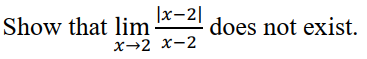Solution: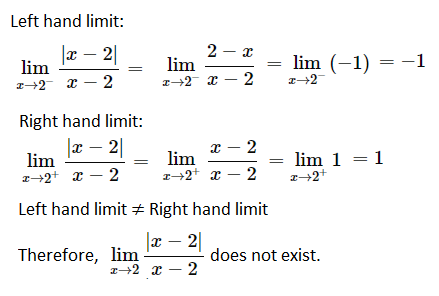(x) Find the point on the curve y2 = 4x, the tangent at which is parallel to the straight line y = 2x + 4.

Solution:

Given curve is:

y2 = 4x….(i)

Equation of the straight line is y = 2x + 4

This is of the form y = mx + c,

Slope = m = 2

Differentiating the equation of given curve,

2y (dy/dx) = 4

dy/dx = (4/2y)

dy/dx = 2/y

We know that the slope of the tangent at a point is given by the value of the derivative of the equation of the curve at that point.

2/y = 2

⇒ y = 1

Substituting y = 1 in (i),

(1)2 = 4x

⇒ x = 1/4

Hence, the required point is (¼, 1).

Question 2

Draw the graph of the function y = |x – 2| + |x – 3|.

Solution:

|x – 2| = x – 2 if x > 2, and |x – 2| = -x + 2 if x < 2

Similarly,

|x – 3| = x – 3 if x > 3, and |x – 3| = -x + 3 if x < 3.

If x < 2, then |x – 2| + |x – 3| = -2x + 5.

If x > 3, then |x – 2| + |x – 3| = 2x – 5.

Now consider another case:

When x is between the values of 2 and 3 then |x – 2| = x – 2 and |x – 3| = -x + 3.

⇒ |x – 2| + |x – 3| = (x – 2) + (-x + 3) = 1

Thus, for x values between 2 and 3, we get the equation y = 1, which is just a horizontal line.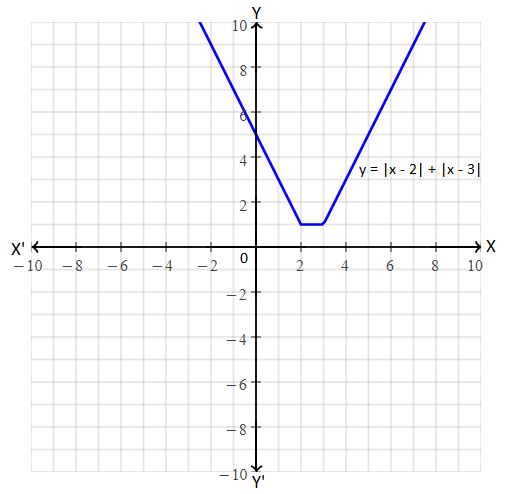Question 3

Prove that cot A + cot(60 + A) + cot(120 + A) = 3 cot 3A.

Solution:

LHS = cot A + cot (60 + A) + cot (120 + A)

= cot A + cot (60 + A) + cot [180 – (60 – A)]

= cot A + cot (60 + A) – cot (60 – A)

= cot A + [(cot 60 cot A – 1)/ (cot 60 + cot A)] – [(cot 60 cot A + 1)/ (cot A – cot 60)]

= cot A + {[(1/√3)cot A – 1]/ [(1/√3) + cot A]} – {[(1/√3) cot A + 1]/ [cot A – (1/√3)]}

= cot A + [(cot A – √3)/ (√3 cot A + 1)] – [(cot A + √3)/ (√3 cot A – 1)]

= cot A + [(cot A – √3)(√3 cot A – 1) – (cot A + √3)(√3 cot A + 1)]/ [(√3 cot A + 1)(√3 cot A – 1)]

= cot A + [(√3 cot2A – cot A – 3 cot A + √3 – √3 cot2A – cot A – 3 cot A – √3)/ (3 cot2A – 1)

= cot A + [(-8 cot A)/ (3 cot2A – 1)

= (3 cot3A – 9 cot A)/ (3 cot2A – 1)

= 3[(cot3A – 3 cot A)/ (3 cot2A – 1)]

= 3 cot 3A

= RHS

Hence proved.

OR

In a ∆ABC prove that b cos C + c cos B = a.

Solution:

Let ABC be a triangle.

Case 1:

ABC is an acute-angled triangle.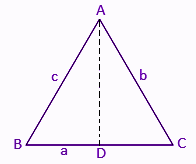a = BC = BD + CD….(i)

cos B = BD/AB

⇒ BD = AB cos B

⇒ BD = c cos B (AB = c)

And

cos C = CD/AC

⇒ CD = AC cos C

⇒ CD = b cos C (AC = b)

From the above,

a = c cos B + b cos C

Case 2:

ABC is a right-angled triangle.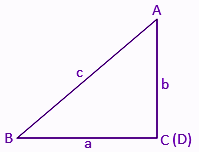a = BC

cos B = BC/AB

⇒ BC = AB cos B

⇒ BC = c cos B, [since, AB = c]

a = c cos B

⇒ a = c cos B + 0

b = c cos A + a cos C

⇒ a = c cos B + b cos C (C = 90° ⇒ cos C = cos 90 = 0)

Case 3:

ABC is an obtuse angle triangle.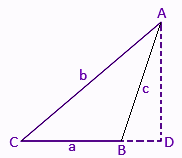a = BC = CD – BD

cos C = CD/AC

⇒ CD = AC cos C

⇒ CD = b cos C (since AC = b)

And,

cos (π – B) = BD/AB

⇒ BD = AB cos (π – B)

a = b cos C + c cos B

⇒ BD = -c cos B [since, AB = c and cos (π – θ) = -cos θ]

From the above,

a = b cos C – (-c cos B)

⇒ a = b cos C + c cos B

Question 4

Find the locus of a complex number, Z = x + iy, satisfying the relation |(z – 3i)/ (z + 3i)| ≤ √2. Illustrate the locus of Z in the argand plane.

Solution:

Given,

z = x + iy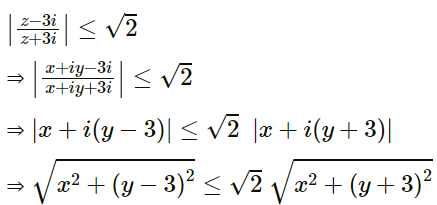Squaring on both sides,

x2 + (y – 3)2 ≤ 2 [x2 + (y + 3)2]

x2 + y2 + 9 – 6y ≤ 2(x2 + y2 + 9 + 6y)

x2 + y2 + 9 – 6y ≤ 2x2 + 2y2 + 18 + 12y

⇒ 2x2 + 2y2 + 18 + 12y – x2 – y2 – 9 + 6y ≥ 9

⇒ x2 + y2 + 18y + 9 ≥ 0

⇒ x2 + (y2 + 18y + 81) + 9 ≥ 81

⇒ x2 + (y + 9)2 ≥ 81 – 9

⇒ x2 + [y – (-9)]2 ≥ 72

⇒ (x – 0)2 + [y – (-9)]2 ≥ (6√2)2

Therefore, the locus of z represents a circle with centre (0, -9) and radius 6√2 units.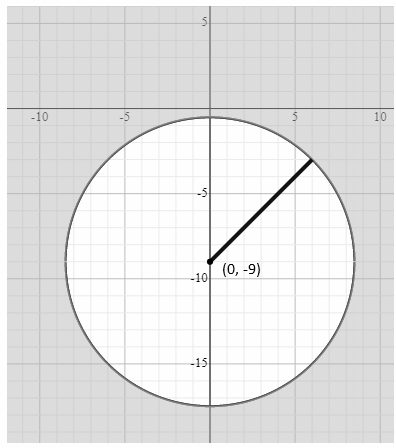Question 5

Find the number of words which can be formed by taking four letters at a time from the word “COMBINATION”.

Solution:

Given word is:

COMBINATION

Number of letters = 11

O – 2 times

I – 2 times

N – 2 times

Thus, 8 distinct letters and 3 letters repeated twice.

Case 1: Selecting 4 different letters as one word

In this case, the number of words can be formed = 8C4 × 4! = 70 × 24 = 1680

Case 2: 2 pairs of repeated letters (for example IIOO)

In this case, the number of words can be formed = 3C2 × (4!/ 2! 2!) = 3 × 6 = 18

Case 3: one pair of repeated letters and 2 different letters (for example NNCB)

In this case, the number of words can be formed = 3C1 × 7C2 × (4!/2!)

= 3 × 21 × 12 = 756

Therefore, the total number of words = 1680 + 18 + 756 = 2454

OR

A committee of 7 members has to be formed from 9 boys and 4 girls. In how many ways can this be done when the committee consists of:

(i) exactly 3 girls

(ii) at least 3 girls and

(iii) at most three girls.

Solution:

Given,

Number of boys = 9

Number of girls = 4

The number of members should be in the committee = 7

(i) Exactly 3 girls

Total number of ways of forming a committee in which exactly 3 girls included = 9C4 × 4C3

= [(9 × 8 × 7 × 6)/ (4 × 3 × 2 × 1)] × 4

= 504

(ii) At least 3 girls

The committee consists of 3 girls + 4 boys and 4 girls + 3 boys

Total number of ways in this case = 4C3 × 9C4 + 4C4 × 9C3

= 504 + 1 × [(9 × 8 × 7)/ (3 × 2 × 1)]

= 504 + 84

= 588

(iii) At most 3 girls

(No girl + 7 boys) or (1 girl + 6 boys) or (2 girls + 5 boys) or (3 girls + 4 boys)

No girl + 7 boys = 4C0 × 9C7 = 1 × 9C2 = (9 × 8)/ (2 × 1) = 36

1 girls + 6 boys = 4C1 × 9C6 = 4 × 9C3 = 4 × [(9 × 8 × 7)/ (3 × 2 × 1)] = 336

2 girls + 5 boys = 4C2 × 9C5 = [(4 × 3)/ (2 × 1)] × 9C4 = 6 × 126 = 756

3 girls + 4 boys = 4C3 × 9C4 = 504

Total number of ways = 36 + 336 + 756 + 504 = 1632

Question 6

Prove by the method of induction.

(1/1.2) + (1/2.3) + (1/3.4) + ………. up to n terms = n/(n + 1) where n ∈ N.

Solution:

Using principle of mathematical induction,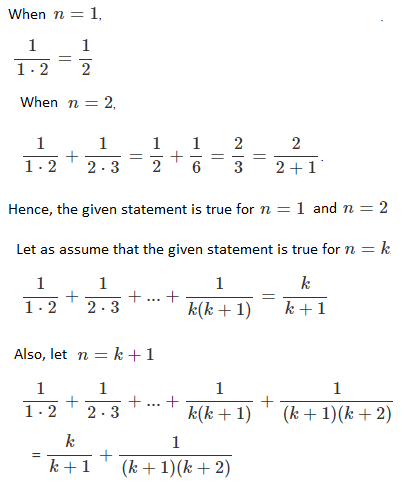= [k(k + 2) + 1]/ [(k + 1)(k + 2)]

= (k2 + 2k + 1)/ (k + 1)(k + 2)

= (k + 1)2/ (k + 1)(k + 2)

= (k + 1)/ (k + 2)

Therefore, the given statement is also true for n = k + 1.

Hence proved that (1/1.2) + (1/2.3) + (1/3.4) + ………. up to n terms = n/(n + 1)

Question 7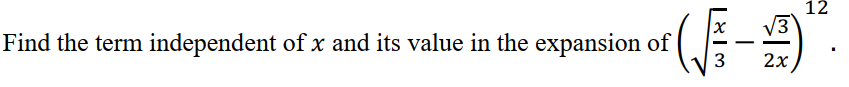Solution:

[(√x/ √3) – (√3/ 2x)]12

We know that the general term of (a + b)n is:

Tr + 1 = nCr (a)n – r (a)r

Tr + 1 = 12Cr (√x/ √3)12 – r . (-√3/ 2x)r

= 12Cr (x/3) (12 – r)/2 . (-3)r/2 [1/ 2r xr]

= 12Cr (3) -(12 – r)/2 (-3)r/2 2-r x[(12 – r)/2 – r]

To get the term which is independent of x, assume the value of power of x as 0.

[(12 – r)/2 – r] = 0

12 – r – 2r = 0

3r = 12

r = 4

Thus, the fourth term will be the required term.

T4 + 1 = 12C4 (3) -(12 – 4)/2 (-3)4/2 2-4

= 12C4 [3-4 (9)]/ 16

= 495 × 9/ (81 × 16)

= 55/16

OR

Find the sum of the terms of the binomial expansion to infinity: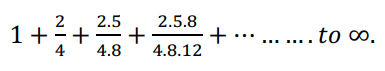Solution: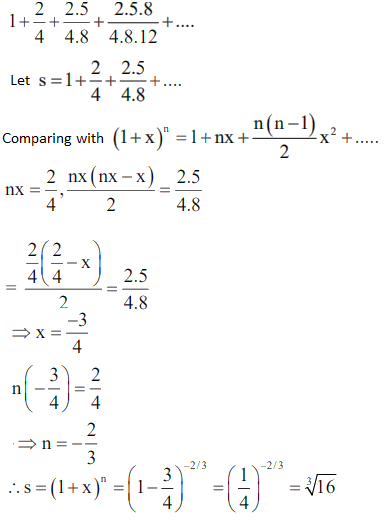Question 8

Differentiate from first principle: f(x) = √(3x + 4).

Solution:

Given,

f(x) = √(3x + 4)

f(x + h) = √[3(x + h) + 4]

By the first principle,

f'(x) = limh → 0 [f(x + h) – f(x)]/ h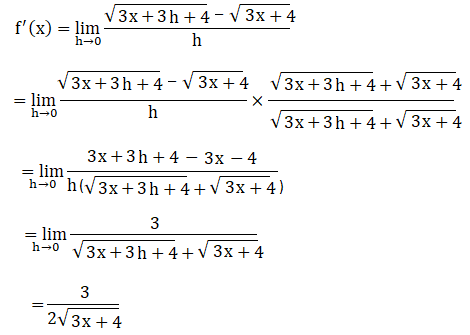Question 9

Reduce the equation x + y + √2 = 0 to the normal form (x cos α + y sin α = p) and find the values of p and α.

Solution:

Given,

x + y + √2 = 0

x + y = -√2

√[(coefficient of x)2 + (coefficient of y)2] = √(12 + 12)

= √(1 + 1)

= √2

⇒ (1/√2)(x + y) = -(1/√2) × √2

⇒ (1/√2)x + (1/√2)y = -1

⇒ x cos (π/4) + y sin (π/4) = -1

Comparing with the normal form x cos α + y sin α = p,

α = π/4 = 45° and p = -1

Therefore, α = 45° and p = -1.

Question 10

Write the equation of the circle having radius 5 and tangent as the line 3x – 4y + 5 = 0 at (1, 2).

Solution:

Let the equation of the circle be:

(x – h)2 + (y – k)2 = r2

(x – h)2 + (y – k)2 = 52 (given radius = 5)

Also, 3x – 4y + 5 = 0 is the tangent to the circle.

⇒ |3h – 4k + 5|/√(32 + 42) = 5

⇒ |3h – 4k + 5|/ √(9 + 16) = 5

⇒ |3h – 4k + 5|/ √25 = 5

⇒ |3h – 4k + 5| = 25

⇒ 3h – 4k + 5 = ±25

Now,

3h – 4k + 5 = 25

3h – 4k = 20….(i)

And

3h – 4k + 5 = -25

3h – 4k = -30….(ii)

Consider, 3x – 4y + 5 = 0

4y = 3x + 5

y = (3/4)x + (5/4)

Slope = (3/4)

Slope of the line passes through radius and the point (1, 2) = -1/slope of the given line

(k – 2)/ (h – 1) = -1/(3/4) = -4/3

3(k – 2) = -4(h – 1)

3k – 6 = -4h + 4

4h + 3k = 10….(iii)

By solving (i), (ii), and (iii),

h = 4, k = -2 ad h = -2 and k = 6

Hence, the required equation of circles are:

(x – 4)2 + (y + 2)2 = 25

(x + 2)2 + (y – 6)2 = 25

Question 11

In a ΔABC, prove that cot A + cot B + cot C = (a2 + b2 + c2)/4Δ.

Solution:

Area of triangle = Δ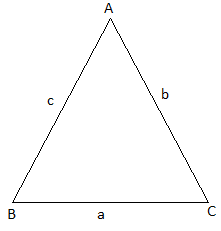We know that in a triangle ABC,

Δ = (1/2) bc sin A

bc = 2Δ/sin A….(i)

Δ = (1/2) ac din B

ac = 2Δ/sin B….(ii)

Δ = (1/2) ab sin C

ab = 2Δ/sin C….(iii)

Using cosine rule,

a2 = b2 + c2 – 2bc cos A

b2 = a2 + c2 – 2ac cos B

c2 = a2 + b2 – 2ab cos C

Now,

a2 + b2 + c2 = 2a2 + 2b2 + 2c2 – 2ab cos C – 2ac cos B – 2bc cos A

⇒ a2 + b2 + c2 = 2ab cos C + 2ac cos B + 2bc cos A….(iv)

From (i), (ii), (iii), and (iv),

a2 + b2 + c2 = 2 [(2Δ/sin C) cos C + (2Δ/sin B) cos B + (2Δ/sin A) cos A]

a2 + b2 + c2 = 2 × 2Δ (cot A + cot B + cot C)

⇒ cot A + cot B + cot C = (a2 + b2 + c2)/4Δ

Hence proved.

Question 12

Find the nth term and deduce the sum to n terms of the series:

4 + 11 + 22 + 37 + 56 + ….

Solution:

Given series is:

4 + 11 + 22 + 37 + 56 + ….

Let Sn = 4 + 11 + 22 + 37 + 56 + … + an – 1 + an (n terms)

⇒ 0 = 4 + 7 + 11 + 15 + 19 + … + (an – an – 1) – an (n + 1 terms)

⇒ an = 4 + 7 + 11 + 15 + 19 + … + (an – an – 1)

7 + 11 + 15 + 19 + … + (an – an – 1) is an AP with a = 7 and d = 4

Sum of these n – 1 terms = (n – 1)/2 [2 × 4 + (n – 1 – 1)4]

= (n – 1)/2 [14 + (n – 2)4]

= (n – 1)[7 + (n – 2)2]

an = 4 + (n – 1)(7 + 2n – 4)

an = 4 + (n – 1)(2n + 3)

= 4 + 2n2 + 3n – 2n – 3

= 2n2 + n + 1

OR

If (p + q)th term and (p – q)th terms of G.P are a and b respectively, prove that the pth term is √(ab).

Solution:

Let t1 be the first term and r be the common ratio of a GP.

Given,

(p + q)th term and (p – q)th terms of G.P are a and b respectively.

t1 × r(p + q – 1) = a….(i)

t1 × r(p – q – 1) = b….(ii)

Multiplying (i) and (ii),

t1 × r(p + q – 1) × t1 × r(p – q – 1) = ab

(t1)2 × r(p + q – 1 + p – q – 1) = ab

(t1)2 × r(2p – 2) = ab

(t1)2 × r2(p – 1) = ab

(t1 × rp – 1)2 = ab

t1 × rp – 1 = √ab

Therefore, pth terms = √ab

Question 13

If x is real, prove that the value of the expression [(x – 1)(x + 3)] / [(x – 2)(x + 4)] cannot be between 4/9 and 1.

Solution:

Let [(x – 1)(x + 3)] / [(x – 2)(x + 4)] = y

(x – 1)(x + 3) = y(x – 2)(x + 4)

x2 + 3x – x – 3 = y(x2 + 4x – 2x – 8)

x2 + 2x – 3 – x2y – 2xy + 8y = 0

x2(1 – y) + 2x(1 – y) + (8y – 3) = 0

Given that x is real.

Therefore, discriminant ≥ 0

[2(1 – y)]2 – 4(1 – y)(8y – 3) ≥ 0

4(1 + y2 – 2y) – 4(8y – 3 – 8y2 + 3y) ≥ 0

4 + 4y2 – 8y – 44y + 12 + 32y2 ≥ 0

36y2 – 52y + 16 ≥ 0

4(9y2 – 13y + 4) ≥ 0

9y2 – 13y + 4 ≥ 0

9y2 – 9y – 4y + 4 ≥ 0

9y(y – 1) – 4(y – 1) ≥ 0

(9y – 4)(y – 1) ≥ 0

(y – 4/9)(y – 1) ≥ 0

 Factor y < 4/9 y ∈ (4/9, 1) y > 1 y – (4/9) -ve +ve +ve y – 1 -ve -ve +ve [y – (4/9)] (y – 1) +ve -ve +ve

[y – (4/9)](y – 1) is positive only when y < 4/9 or y > 1.

Therefore, y cannot lie between 4/9 and 1.

OR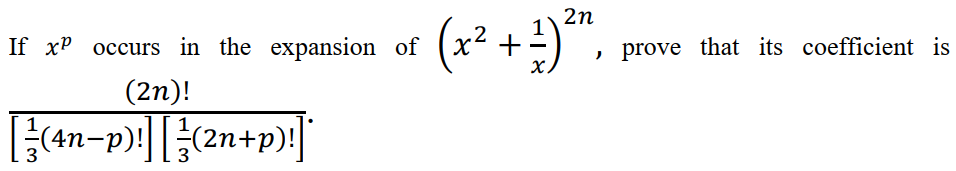Solution:

Given,

[x2 + (1/x)]2n

General term is:

Tr + 1 = 2nCr (x2)2n – r (1/x)r

= 2nCr x4n – 2r x(-r)

= 2nCr x(4n – 2r – r)

= 2nCr x(4n – 3r)

Let xp occur in this expansion.

⇒ 4n – 3r = p

⇒ 3r = 4n – p

⇒ r = (4n – p)/3

Coefficient of xp = 2nCr

= (2n)!/ [r!(2n – r)!]

= (2n)!/ {[(4n – p)/3]! [2n – (4n – p)/3]!}

= (2n)!/ {[(4n – p)/3]! [(6n – 4n + p)/3]!}

= (2n)!/ {[(4n – p)/3]! [(2n + p)/3]!}

Hence proved.

Question 14

Calculate the standard deviation of the following distribution:

 Age 20 – 25 25 – 30 30 – 35 35 – 40 40 – 45 45 – 50 No. of persons 170 110 80 45 40 35

Solution:

 Class Frequency (fi) Class mark (xi) fixi fi(xi)2 20 – 25 170 22.5 3825 86062.5 25 – 30 110 27.5 3025 83187.5 30 – 35 80 32.5 2600 84500 35 – 40 45 37.5 1687.5 63281.25 40 – 45 40 42.5 1700 72250 45 – 50 35 47.5 1662.5 78968.75 Total ∑fi = 480 ∑fixi = 14500 ∑fi(xi)2 = 468250

Variance = 1/(N – 1) [∑fixi2 – (1/N) (∑fixi)2]

= [1/(480 – 1)] [468250 – (14500)2/ 480]

= (1/479) [468250 – 438020.833]

= 30229.167/ 479

= 63.109

Standard deviation = √(63.109) = 7.944

SECTION B

Question 15

(a) Find the focus and directrix of the conic represented by the equation 5x2 = -12y.

Solution:

5x2 = -12y

x2 = (-12/5)y

x2 = 4(-3/5)y

(x – 0)2 = 4(-3/5) (y – 0)

Comparing with (x – h)2 = 4p(y – k)

h = 0, k = 0, p = -3/5

Thus, the given equation represents a parabola with vertex at (h, k) = (0, 0) and focal length |p| = 3/5.

Here, the parabola is symmetric around the y-axis.

Therefore, the focus lies at a distance p from the centre (0, 0) along the y-axis.

i.e. (0, 0 + p)

= (0, 0 + (-3/5))

= (0, -3/5)

Since the parabola is symmetric around the y-axis, the directrix is a line parallel to the x-axis at a distance -p from the centre (0, 0).

Directrix is y = 0 – p

y = 0 – (-3/5)

y = 3/5

Hence, the focus of the given conic is (0, -3/5) and the directrix is y = 3/5.

(b) Construct the truth table (~p ⋀ ~q) ⋁ (p ⋀ ~q)

Solution:

 p q ~p ~q ~p ⋀ ~q p ⋀ ~q (~p ⋀ ~q) ⋁ (p ⋀ ~q) T T F F F F F T F F T F T T F T T F F F F F F T T T F T

(c) Write the converse, contradiction and contrapositive of the statement.

“If x + 3 = 9, then x = 6.”

Solution:

Given statement is:

If x + 3 = 9, then x = 6.

Converse of the given conditional statement is:

If x = 6, then x + 3 = 9.

Contradiction of the given conditional statement is:

If x + 3 ≠ 9, then x ≠ 6.

Contrapositive statement is:

If x ≠ 6, then x + 3 ≠ 9.

Question 16

Show that the point (1, 2, 3) is common to the lines which join A(4, 8, 12) to B(2, 4, 6) and C(3, 5, 4) to D(5, 8, 5).

Solution:

Given,

A(4, 8, 12), B(2, 4, 6), C(3, 5, 4) and D(5, 8, 5).

Let P = (1, 2, 3)

P is a common point to the line AB and CD if ABP and CDP are collinear.

Consider A(4, 8, 12), B(2, 4, 6) and P(1, 2, 3):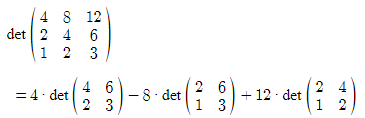= 4(12 – 12) – 8(6 – 6) + 12(4 – 4)

= 4(0) – 8(0) + 12(0)

= 0

Thus, A, B, and P are collinear.

Consider C(3, 5, 4), D(5, 8, 5) and P(1, 2, 3):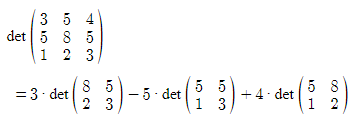= 3(24 – 10) – 5(15 – 5) + 4(10 – 8)

= 3(14) – 5(10) + 4(2)

= 42 – 50 + 8

= 0

Thus, C, D, and P are collinear.

Therefore, the point (1, 2, 3) is common to the lines which join A(4, 8, 12) to B(2, 4, 6) and C(3, 5, 4) to D(5, 8, 5).

OR

Calculate the Cosine of the angle A of the triangle with vertices A(1, -1, 2) B (6, 11, 2) and C(1, 2, 6).

Solution:

Given,

Vertices of a triangle are A(1, -1, 2) B (6, 11, 2) and C(1, 2, 6).

Using distance formula,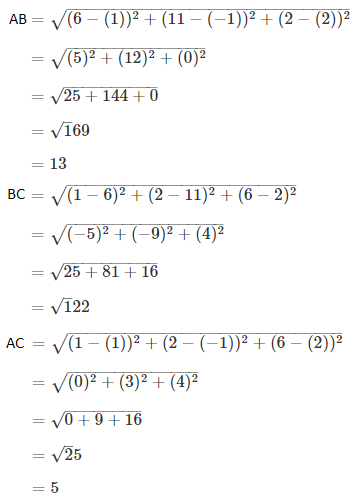AB = c = 13

BC = a = √122

AC = b = 5

cos A = (b2 + c2 – a2)/2bc

= (25 + 169 – 122)/ (2 × 5 × 13)

= 72/130

= 36/65

Question 17

Find the equation of the hyperbola whose focus is (1, 1), the corresponding directrix 2x + y – 1 = 0 and e = √3.

Solution:

Given,

Focus = S(1, 1)

Directrix is 2x + y – 1 = 0

Eccentricity (e) = √3

Let P(x, y) be any point on the hyperbola.

We know that,

The perpendicular distance from the point (x1, y1) to the line ax + by + c = 0 = |ax1 + by1 + c|/ √(a2 + b2)

Here,

(x1, y1) = (x, y)

a = 2, b = 1, c = -1

And

SP = e (perpendicular distance)

SP2 = e2 (perpendicular distance)2

(x – 1)2 + (y – 1)2 = (√3)2 [|2x + y – 1|/ √(22 + 12)]2

x2 – 2x + 1 + y2 – 2y + 1 = (3) [|2x + y – 1|2/ (4 + 1)]

x2 + y2 – 2x – 2y + 2 = (3/5) (4x2 + y2 + 1 + 4xy – 2y – 4x)

5x2 + 5y2 – 10x – 10y + 10 = 12x2 + 3y2 + 3 + 12xy – 6y – 12x

12x2 + 3y2 + 3 + 12xy – 6y – 12x – 5x2 – 5y2 + 10x + 10y – 10 = 0

7x2 – 2y2 + 12xy – 2x + 4y – 7 = 0

Therefore, the equation of the hyperbola is 7x2 – 2y2 + 12xy – 2x + 4y – 7 = 0.

OR

Find the equation of tangents to the ellipse 4x2 + 5y2 = 20 which are perpendicular to the line 3x + 2y – 5 = 0.

Solution:

Given,

4x2 + 5y2 = 20

(4x2/20) + (5y2/20) = 1

(x2/5) + (y2/4) = 1

This is of the form (x2/a2) + (y2/b2) = 1

a2 = 5 and b2 = 4

Equation of the line is 3x + 2y – 5 = 0

2y = -3x + 5

y = (-3/2)x + (5/2)

Slope = -3/2

Tangent and the given equation are perpendicular lines.

Thus, the slope of tangent line = m = -1/(-3/2) = ⅔

Equation of tangent is:

y = mx ± √(a2m2 + b2)

y = (2/3)x ± √[5(4/9) + 4]

y = (2/3)x ± √[(20 + 36)/9

y = (1/3) [2x ± √56]

3y = 2x ± √56

Question 18

Show that the equation 16x2 – 3y2 – 32x – 12y – 44 = 0 represents a hyperbola. Find the lengths of axes and eccentricity.

Solution:

Given,

16x2 – 3y2 – 32x – 12y – 44 = 0

(16x2 – 32x) – (3y2 + 12y) – 44 = 0

16(x2 – 2x) – 3(y2 + 4y) – 44 = 0

16(x2 – 2x + 1) – 3(y2 + 4y + 4) – 44 = 16 – 12

16(x – 1)2 – 3(y + 2)2 – 44 – 4 = 0

16(x – 1)2 – 3(y + 2)2 = 48

⇒ [(x – 1)2/ 3] – [(y + 2)2/ 16] = 1

This is the equation of parabola in which a2 = 3 and b2 = 16.

Centre = (1, -2)

And

b2 = a2(e2 – 1)

16 = 3(e2 – 1)

e2 – 1 = 16/3

e2 = (16/3) + 1

e2 = 19/3

e = √(19/3)

SECTION C

Question 19

(i) Two sample sizes of 50 and 100 are given.The mean of these samples respectively are 56 and 50. Find the mean of size 150 by combining the two samples.

Solution:

Let n1 and n2 be the sizes of two samples.

X1 and X2 are the means of two samples.

According to the given,

n1 = 50 and n2 = 100

X1 = 56 and X2 = 50

New sample size = n1 + n2 = 50 + 100 = 150

New mean = (n1X1 + n2X2)/ (n1 + n2)

= [50(56) + 100(50)]/ 150

= (2800 + 5000)/ 150

= 7800/150

= 52

Therefore, the mean of size 150 by combining the two samples is 52.

(ii) Calculate P95, for the following data:

 Marks 0 – 10 10 – 20 20 – 30 30 – 40 40 – 50 50 – 60 Frequency 3 7 11 12 23 4

Solution:

 Marks (C.I) Frequency Cumulative frequency 0 – 10 3 3 10 – 20 7 10 20 – 30 11 21 30 – 40 12 33 40 – 50 23 56 50 – 60 4 60

N = 60

P at 95 = p = (95/100) × 60 = 57

Cumulative frequency greater than and nearest to 57 is 60 which lies in the interval 50 – 60.

Percentile class = 50 – 60

Lower limit of the percentile class = l = 50

Frequency of the percentile class = f = 4

Cumulative frequency of the class preceding the percentile class = cf = 56

Class height = h = 10

P95 = l + [(p – cf)/ f] × h

= 50 + [(57 – 56)/ 4] × 10

= 50 + (10/4)

= 50 + 2.5

= 52.5

OR

Calculate mode for the following data.

 C.I. 17 – 19 14 – 16 11 – 13 8 – 10 5 – 7 2 – 4 Frequency 12 4 8 16 11 4

Solution:

Let us arrange the data as shown below:

 C.I Frequency 1.5 – 4.5 4 4.5 – 7.5 11 7.5 – 10.5 16 10.5 – 13.5 8 13.5 – 16.5 4 16.5 – 19.5 12

From the given data,

Maximum frequency = 16

Modal class is 7.5 – 10.5

Frequency of the modal class = f1 = 16

Frequency of the class preceding the modal class = f0 = 11

Frequency of the class succeeding the modal class = f2 = 8

Lower limit of the modal class = l = 7.5

Class height = h = 3

Mode = l + [(f1 – f0)/ (2f1 – f0 – f2)] × h

= 7.5 + [(16 – 11)/ (2 × 16 – 11 – 8)] × 3

= 7.5 + [5/ (32 – 19)] × 3

= 7.5 + (15/ 13)

= 7.5 + 1.154

= 8.654

Question 20

(i) Find the covariance between X and Y when N = 10, ∑X = 50, ∑Y = -30, and ∑XY = 115.

Solution:

Given,

N = 10,

∑X = 50

∑Y = -30

∑XY = 115

Covariance between X and Y = (1/N) ∑XY – (1/N2) ∑X ∑Y

= (1/10) × 115 – (1/100) × 50 × (-30)

= 11.5 + 15

= 26.5

(ii) Calculate Spearman’s Rank Correlation for the following data and interpret the result:

 Marks in Mathematics 36 48 27 36 29 30 36 39 42 48 Marks in Statistics 27 45 24 27 31 33 35 45 41 45

Solution:

 Mathematics Rank (R1) Statistics Rank (R2) d = R1 – R2 d2 36 6 27 8.5 -2.5 6.25 48 1.5 45 2 0.5 0.25 27 10 24 10 0 0 36 6 27 8.5 -2.5 6.25 29 9 31 7 2 4 30 8 33 6 2 4 36 6 35 5 1 1 39 4 45 2 2 4 42 3 41 4 -1 1 48 1.5 45 2 -0.5 0.25

n = 10

∑d2 = 27

The spearman’s rank correlation coefficient = 1 – [(6 × ∑d2)/ n(n2 – 1)]

= 1 – [(6 × 27)/ 10(100 – 1)]

= 1 – [162/ 10 × 99]

= 1 – (162/990)

= (990 – 162)/ 990

= 828/990

= 0.836

OR

Find Karl Pearson’s Correlation Coefficient from the given data:

 x 21 24 26 29 32 43 25 30 35 37 y 120 123 125 128 131 142 124 129 134 136

Solution:

 x (x – mean) (x – 30.2) dx d2x y (y – mean) (y – 129.2) dy d2y dx dy 21 -9.2 84.64 120 -9.2 84.64 84.64 24 -6.2 38.44 123 -6.2 38.44 38.44 26 -4.2 17.64 125 -4.2 17.64 17.64 29 -1.2 1.44 128 -1.2 1.44 1.44 32 1.8 3.24 131 1.8 3.24 3.24 43 12.8 163.84 142 12.8 163.84 163.84 25 -5.2 27.04 124 -5.2 27.04 27.04 30 -0.2 0.04 129 -0.2 0.04 0.04 35 4.8 23.04 134 4.8 23.04 23.04 37 6.8 46.24 136 6.8 46.24 46.24 ∑x = 302 ∑dx = 0 ∑d2x = 405.6 ∑y = 1292 ∑dy = 0 ∑d2y = 405.6 ∑dx dy = 405.6

Mean of x = ∑x/n = 302/10 = 30.2

Mean of y = ∑y/n = 1292/10 = 129.2

Karl Pearson’s Correlation Coefficient = (∑dx dy)/ (√∑d2x ∑d2y)

= 405.6 / √(405.6 × 405.6)

= 405.6/405.6

= 1

Question 21

Find the consumer price index for 2007 on the basis of 2005 from the following data using weighted average of price relative method:

 Items Food Rent Cloth Fuel Prive in 2005 (Rs.) 20 100 150 50 Price in 2007 (Rs.) 280 200 120 100 Weighted 30 20 20 10

Solution:

 Items Weights (w) Year 2005 (p0) Year 2007 (p1) Price relative I = (p1/p0) × 100 Iw Food 30 20 280 1400 42000 Rent 20 100 200 200 4000 Cloth 20 150 120 80 1600 Fuel 10 50 100 200 2000 Total ∑w = 80 ∑Iw = 49600

Index number of weighted average of price relatives = ∑Iw/ ∑w

= 49600/ 80

= 620

Question 22

Using the following data. Find out the trend using Quarterly moving average and plot them on graph:

 Year/ Quarter Q1 Q2 Q3 Q4 1994 29 37 43 34 1995 90 42 55 43 1996 47 51 63 53

Solution:

 Year Quarter Quarterly moving total Quarterly moving average Centered moving average (trend values) 1994 I 29 1994 II 37 143 35.75 1994 III 43 43.375 204 51 1994 IV 34 51.625 209 52.25 1995 I 90 53.75 221 55.25 1995 II 42 56.375 230 57.5 1995 III 55 52.125 187 46.75 1995 IV 43 47.875 196 49 1996 I 47 50 204 51 1996 II 51 52.25 214 53.5 1996 III 63 1996 IV 53

Trend: